# Selina Solutions Concise Maths Class 7 Chapter 3: Fraction (Including Problems)

Selina Solutions Concise Maths Class 7 Chapter 3 Fraction (Including Problems) gives students a strong knowledge about the basic concepts. Students are advised to practise the chapter wise problems in order to score well in the annual exam. The expert faculty at BYJU’S have curated the solutions by keeping in mind the understanding abilities of students. The solutions are available in simple PDF format, which can be downloaded based on their requirements. Selina Solutions Concise Maths Class 7 Chapter 3 Fraction (Including Problems) PDF links are available here, for free download.

Chapter 3 covers concepts like classification of fractions, reducing the given fraction to its lowest term, simple and complex fraction, like and unlike fractions, comparing fraction and mathematical operations on fraction. The solutions contain explanations in a step wise manner, to help students gain a better understanding of the concepts.

## Selina Solutions Concise Maths Class 7 Chapter 3: Fraction (Including Problems) Download PDF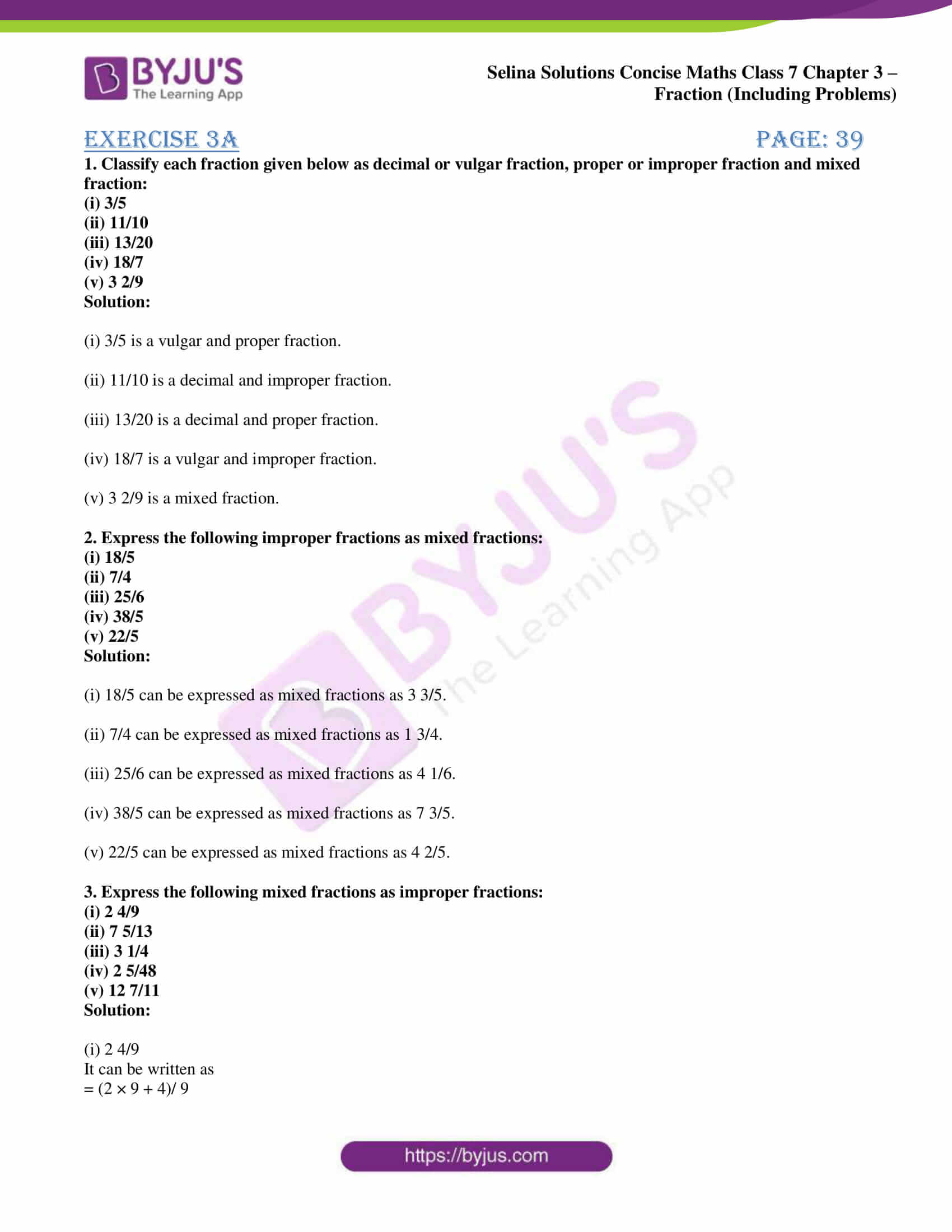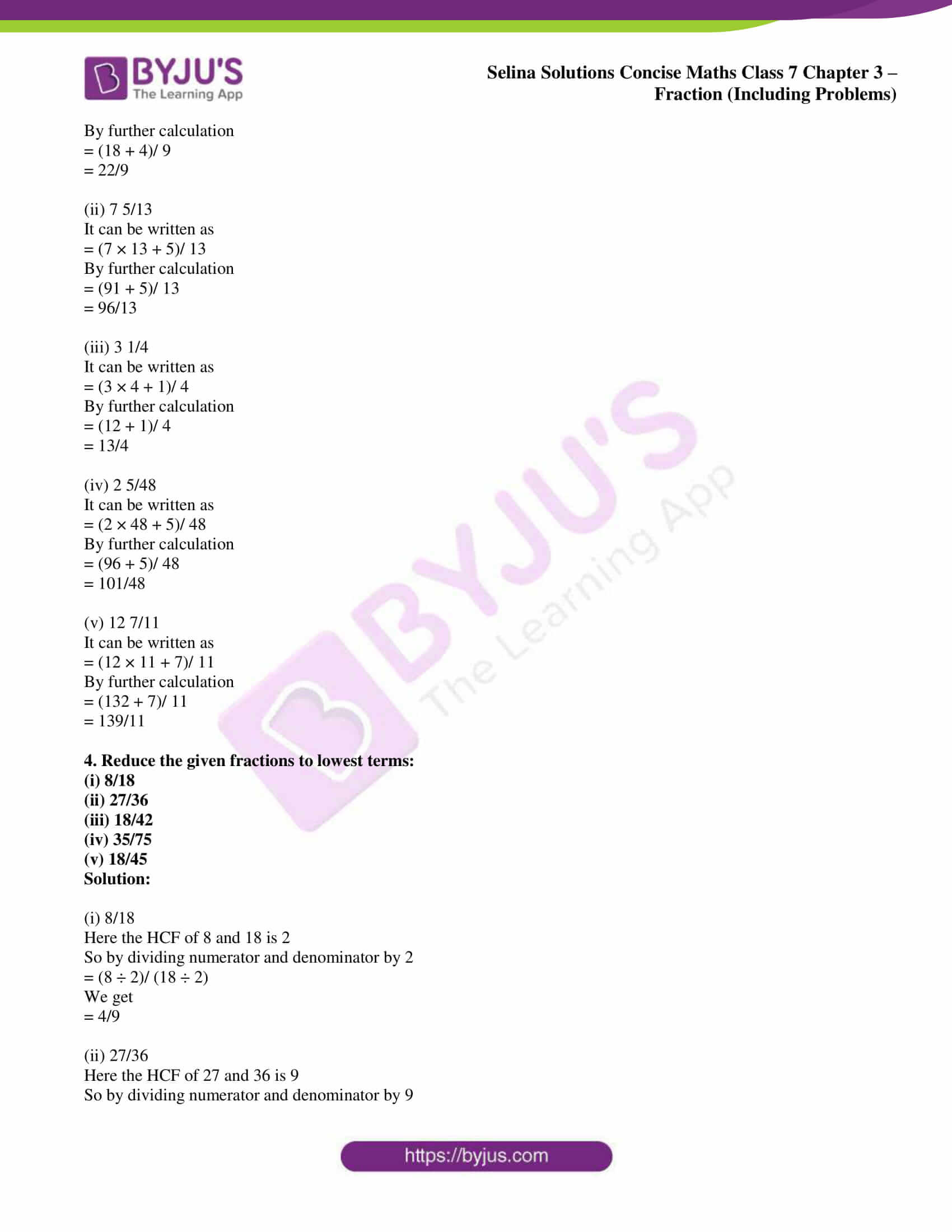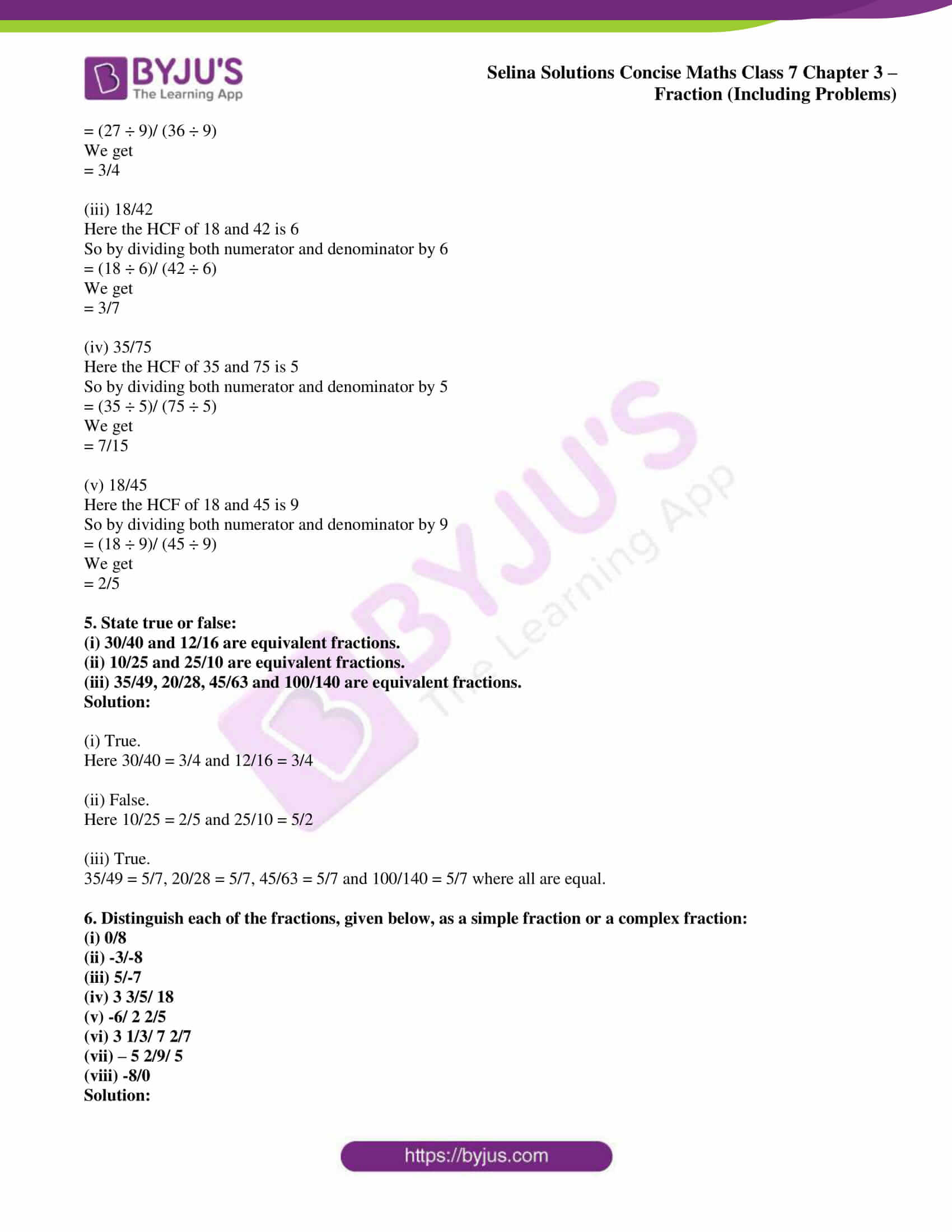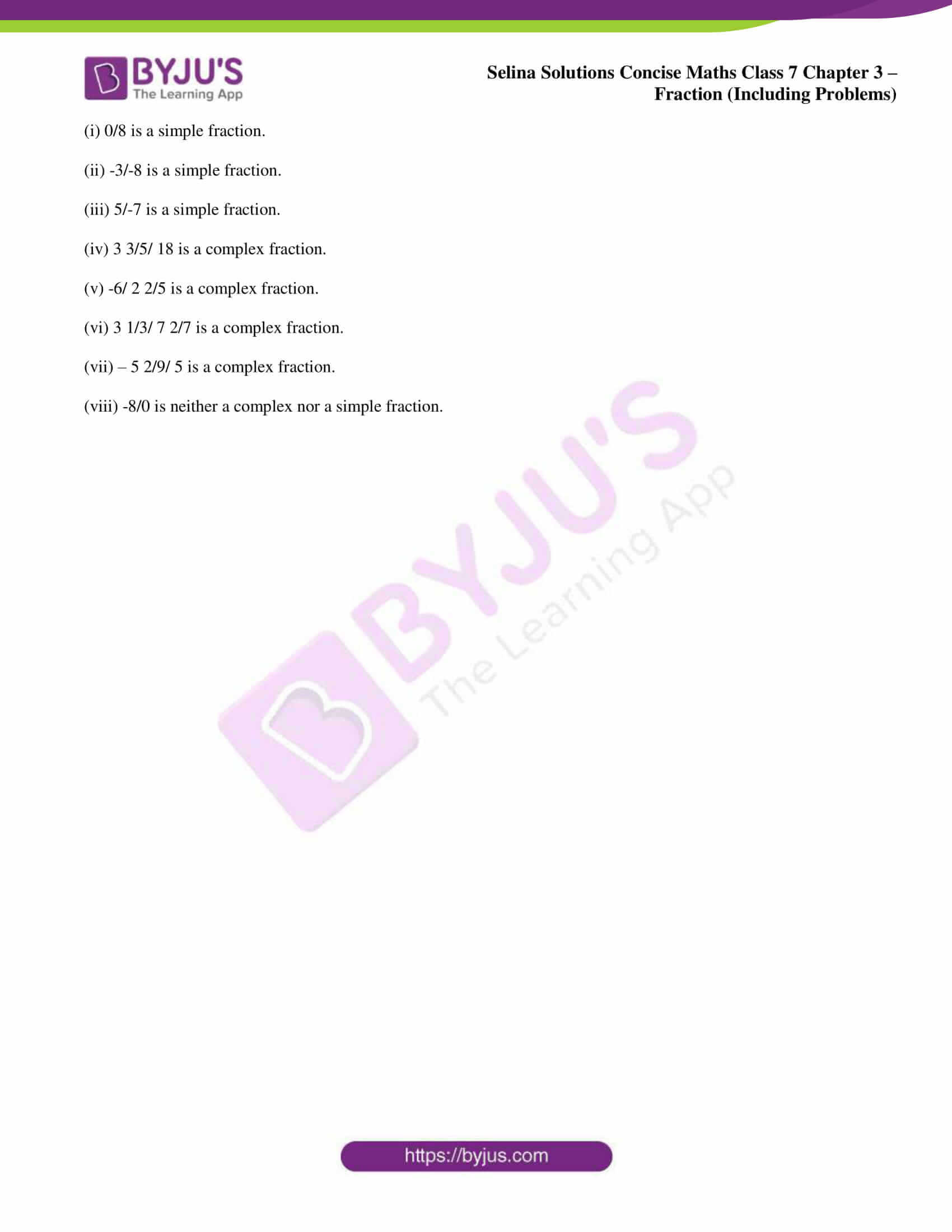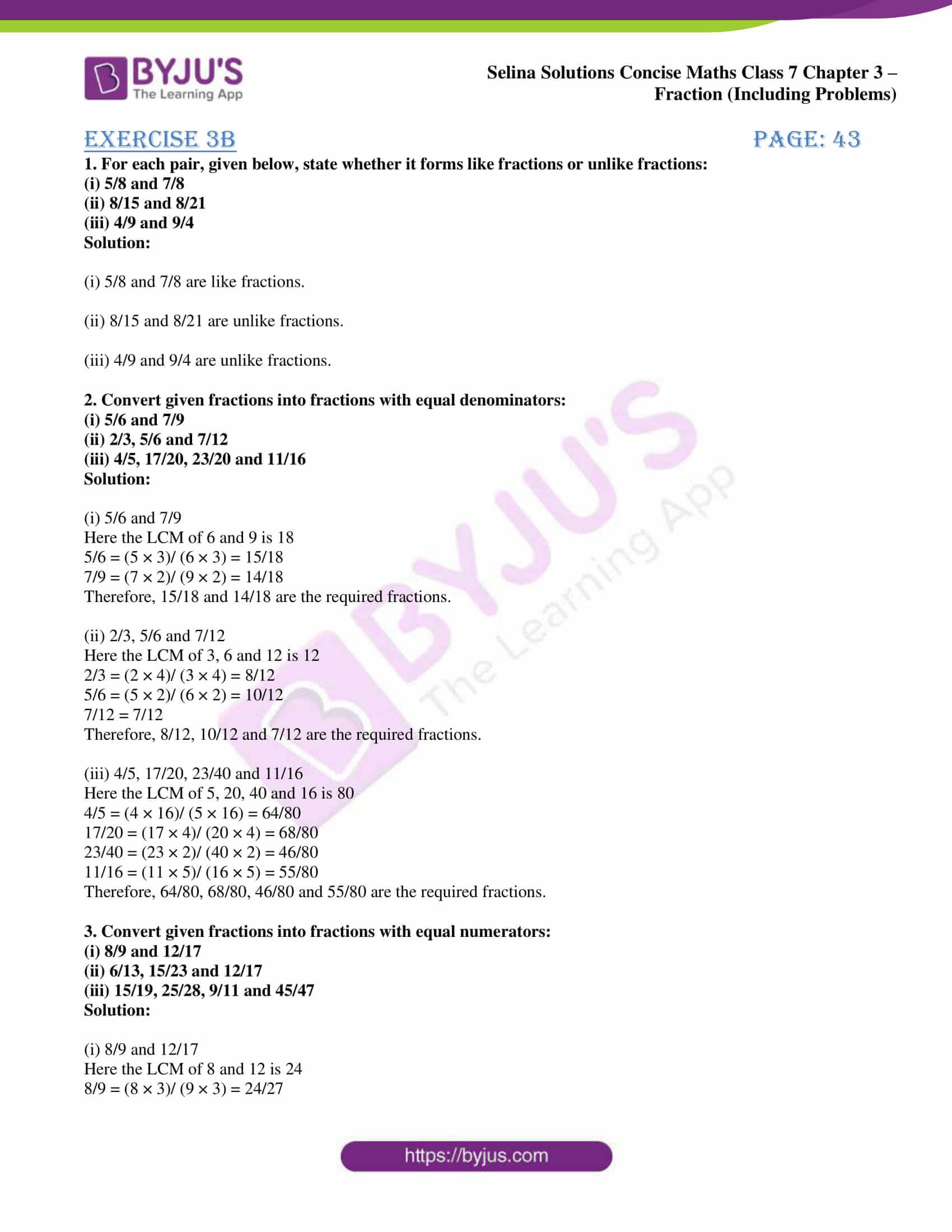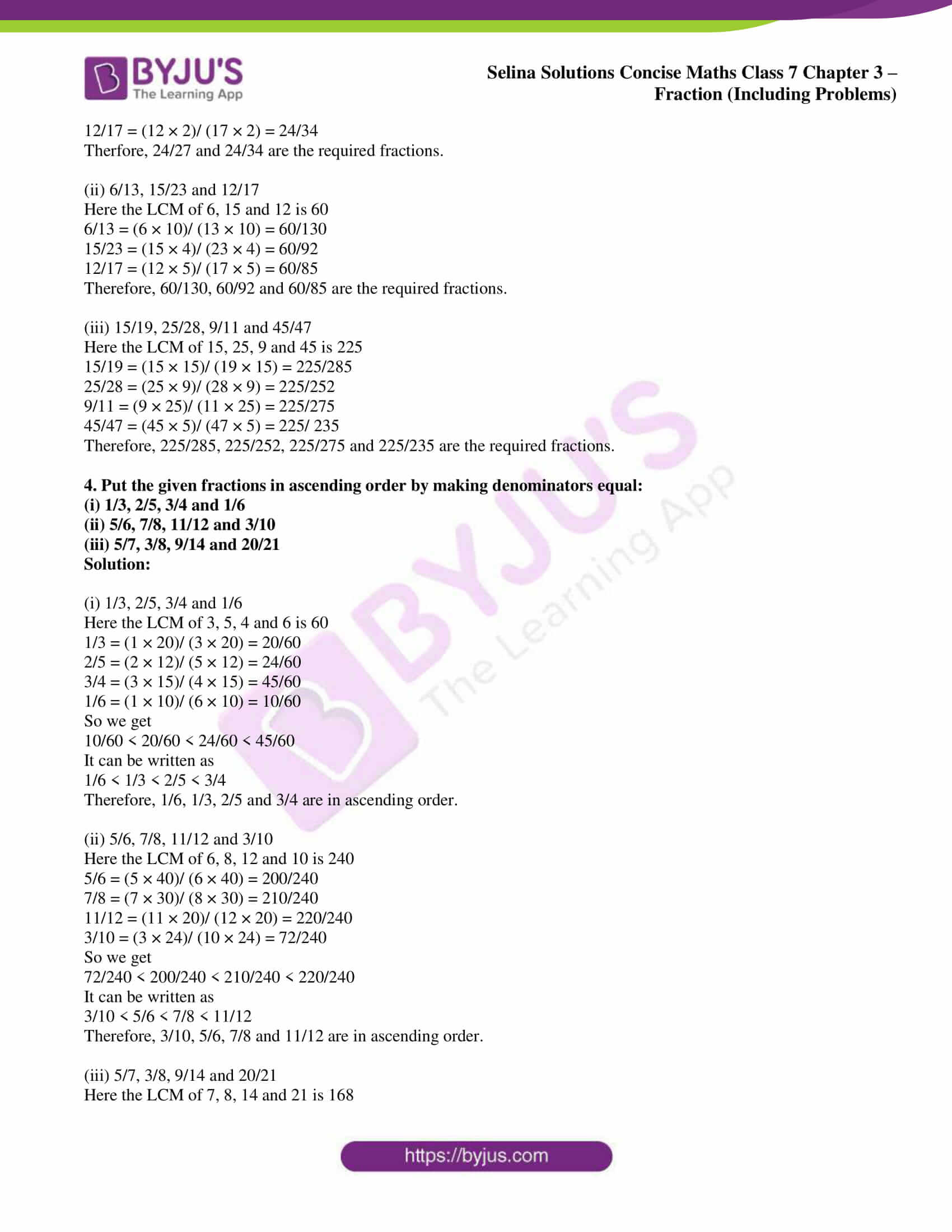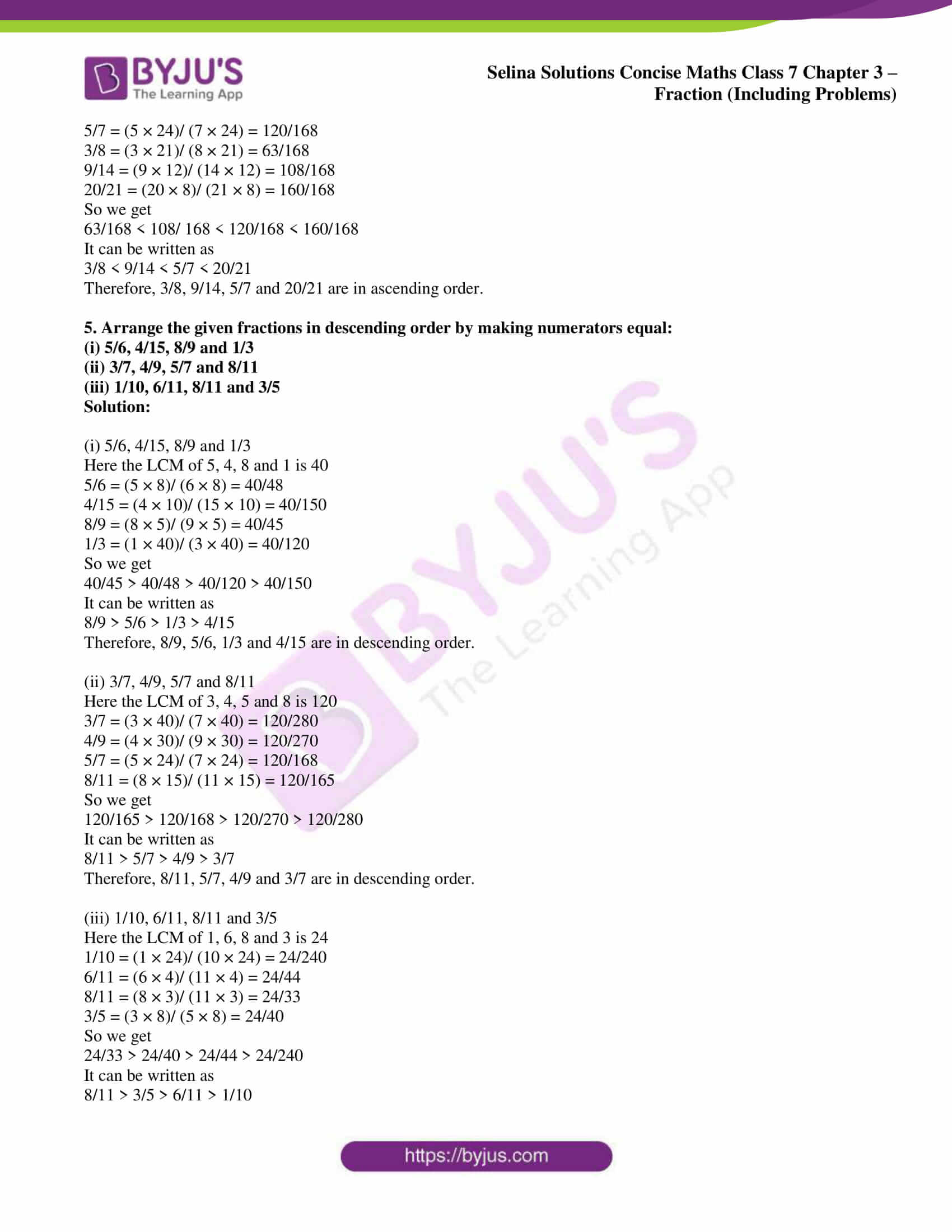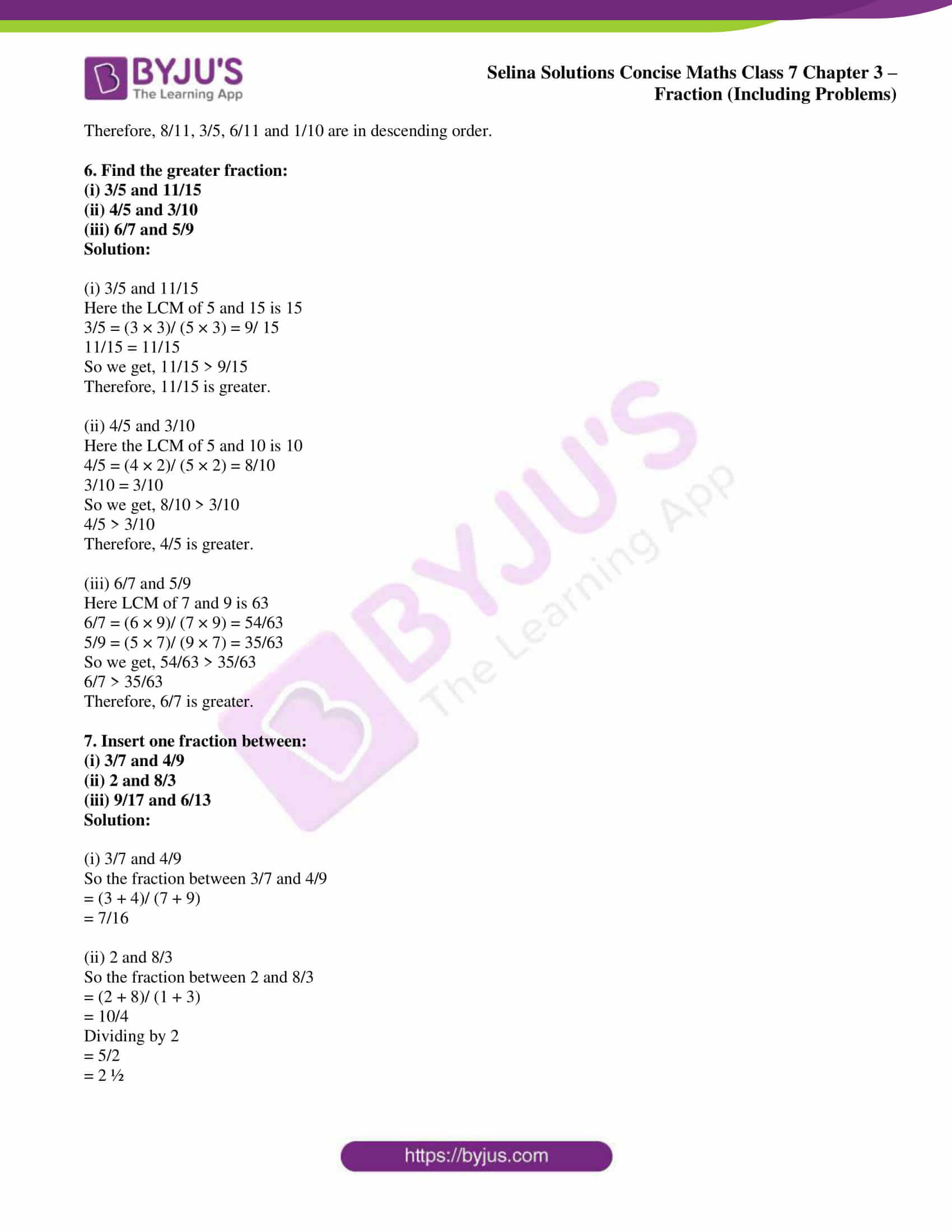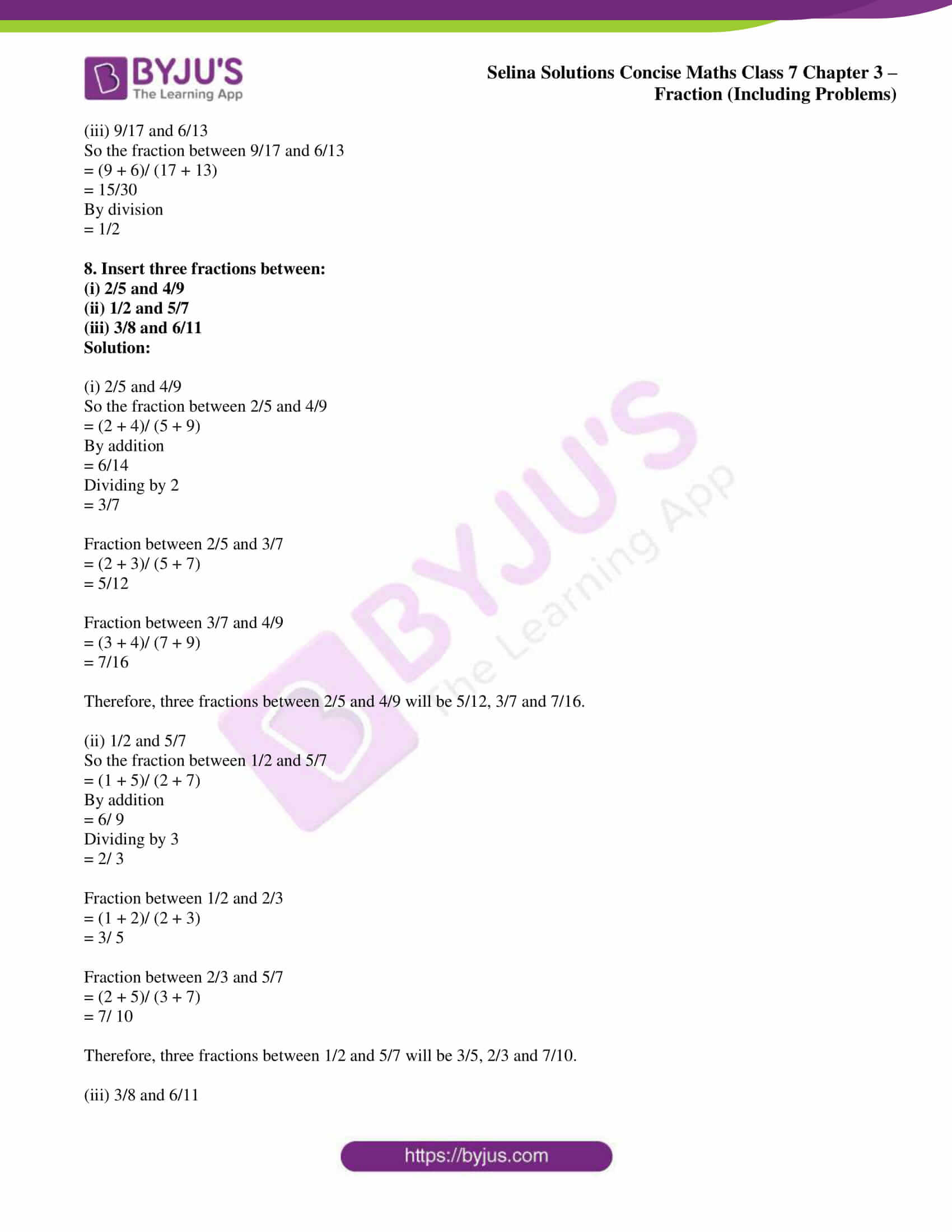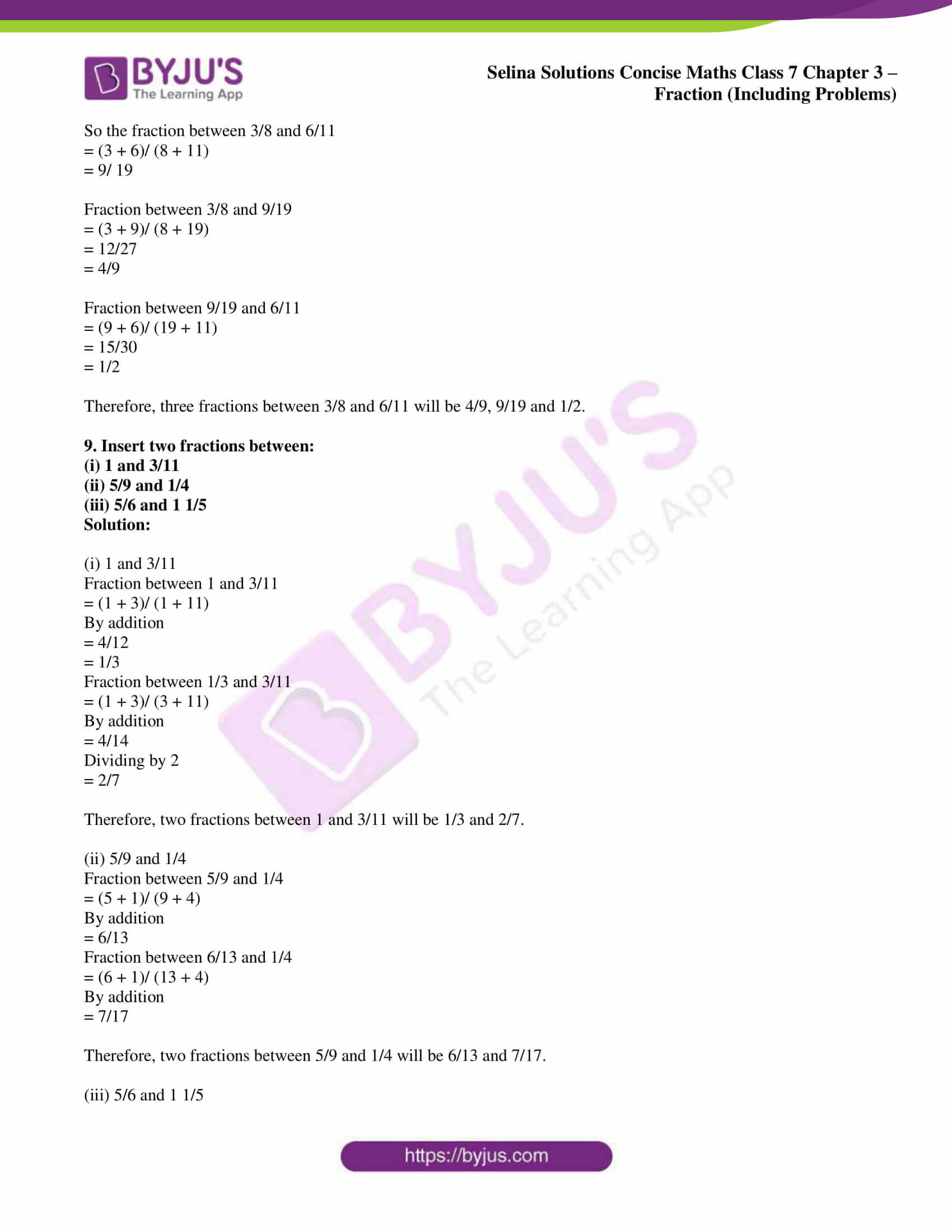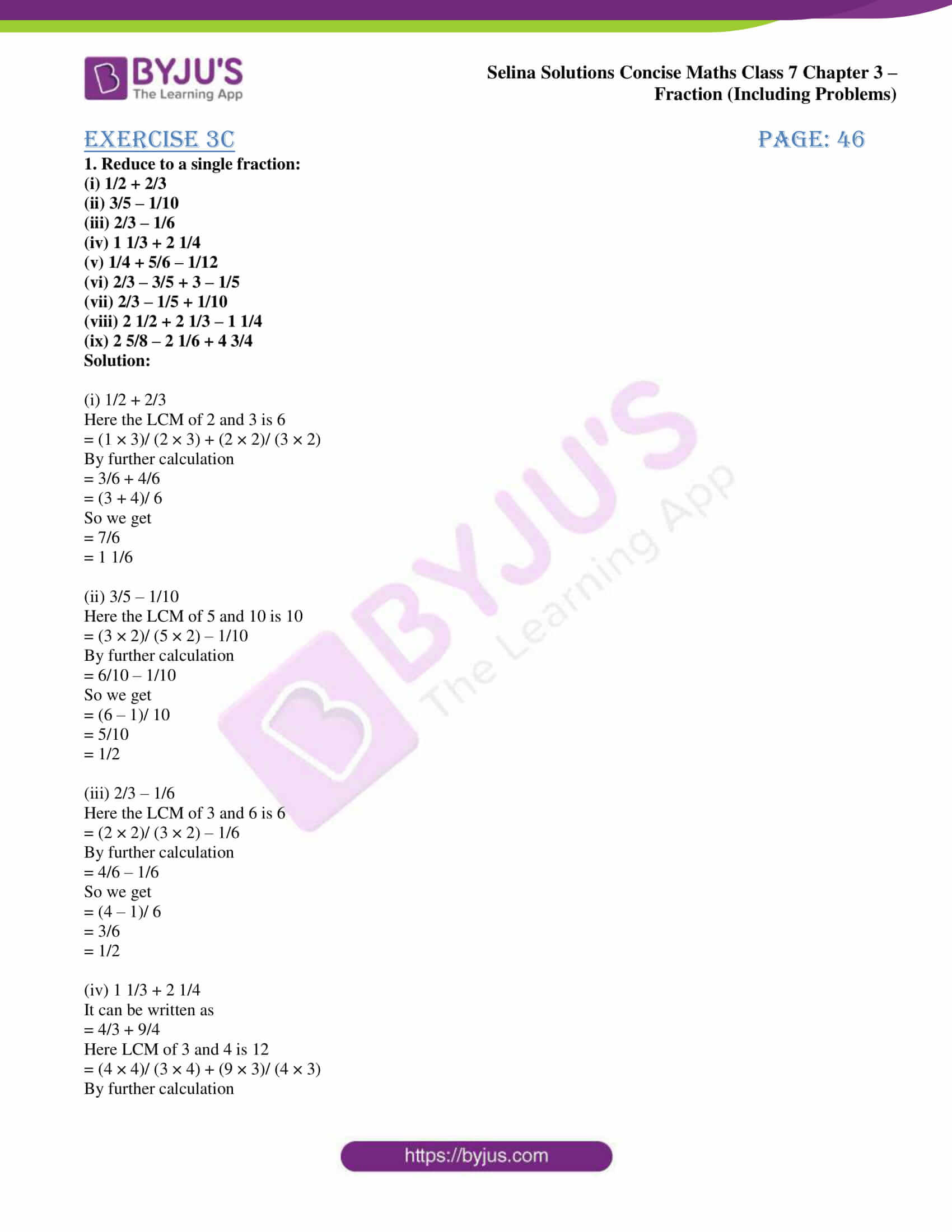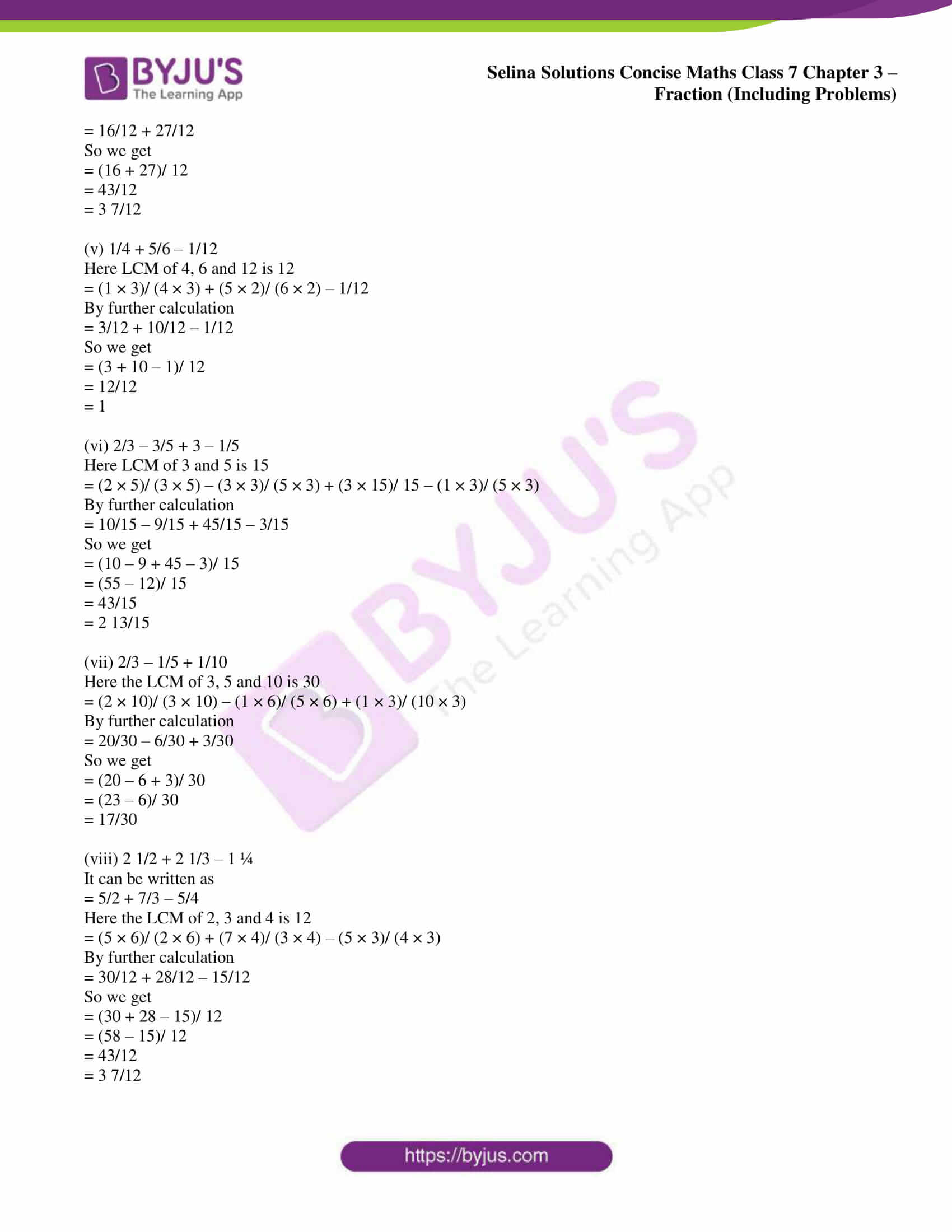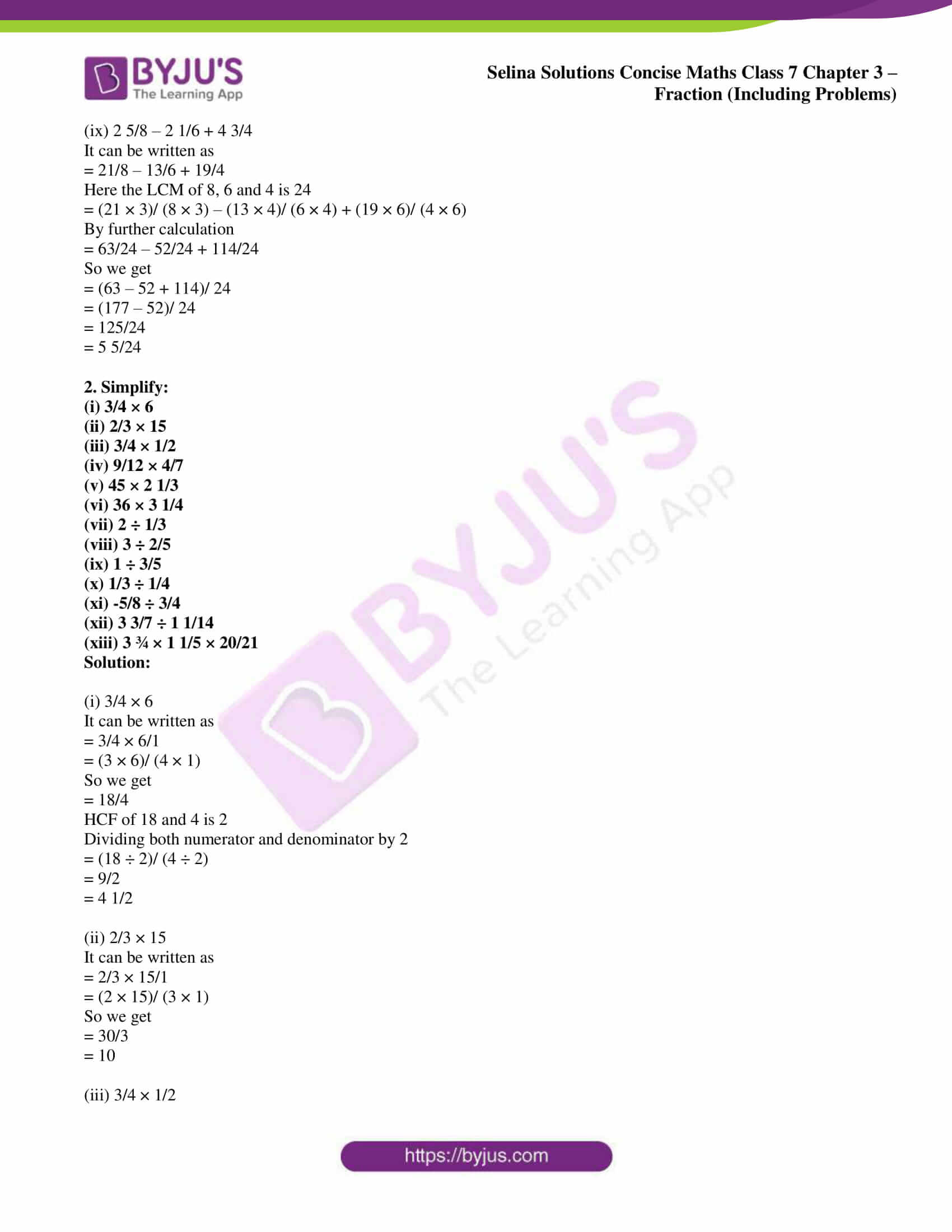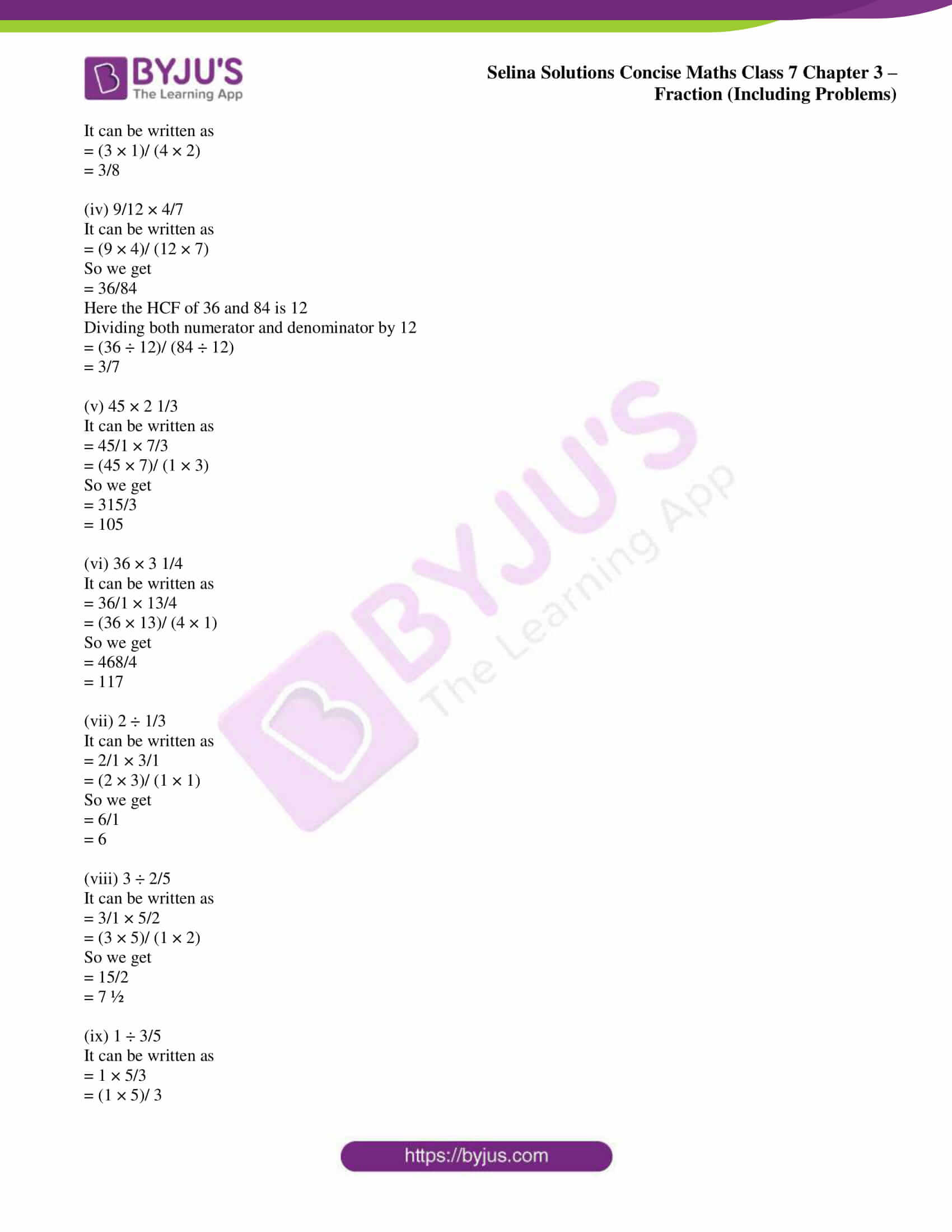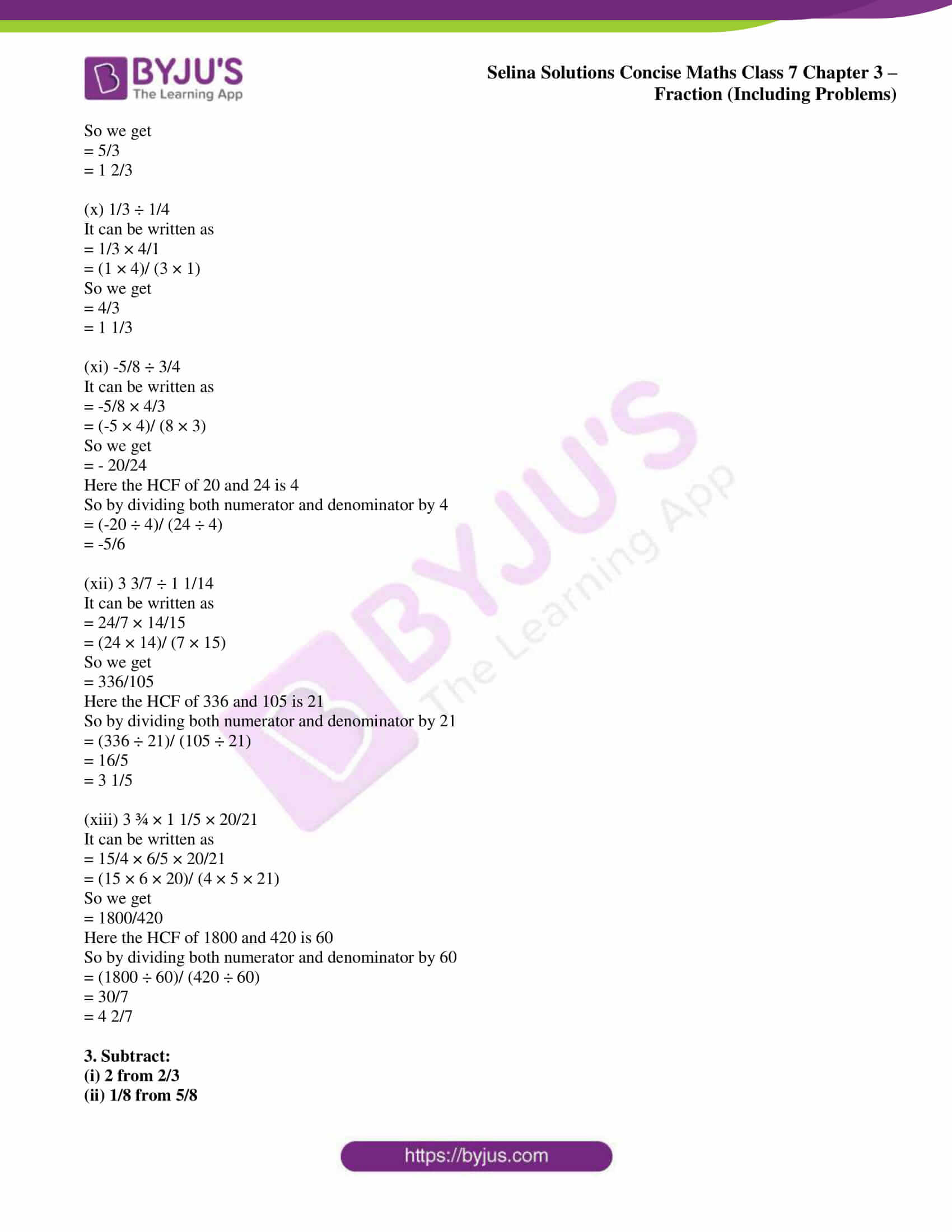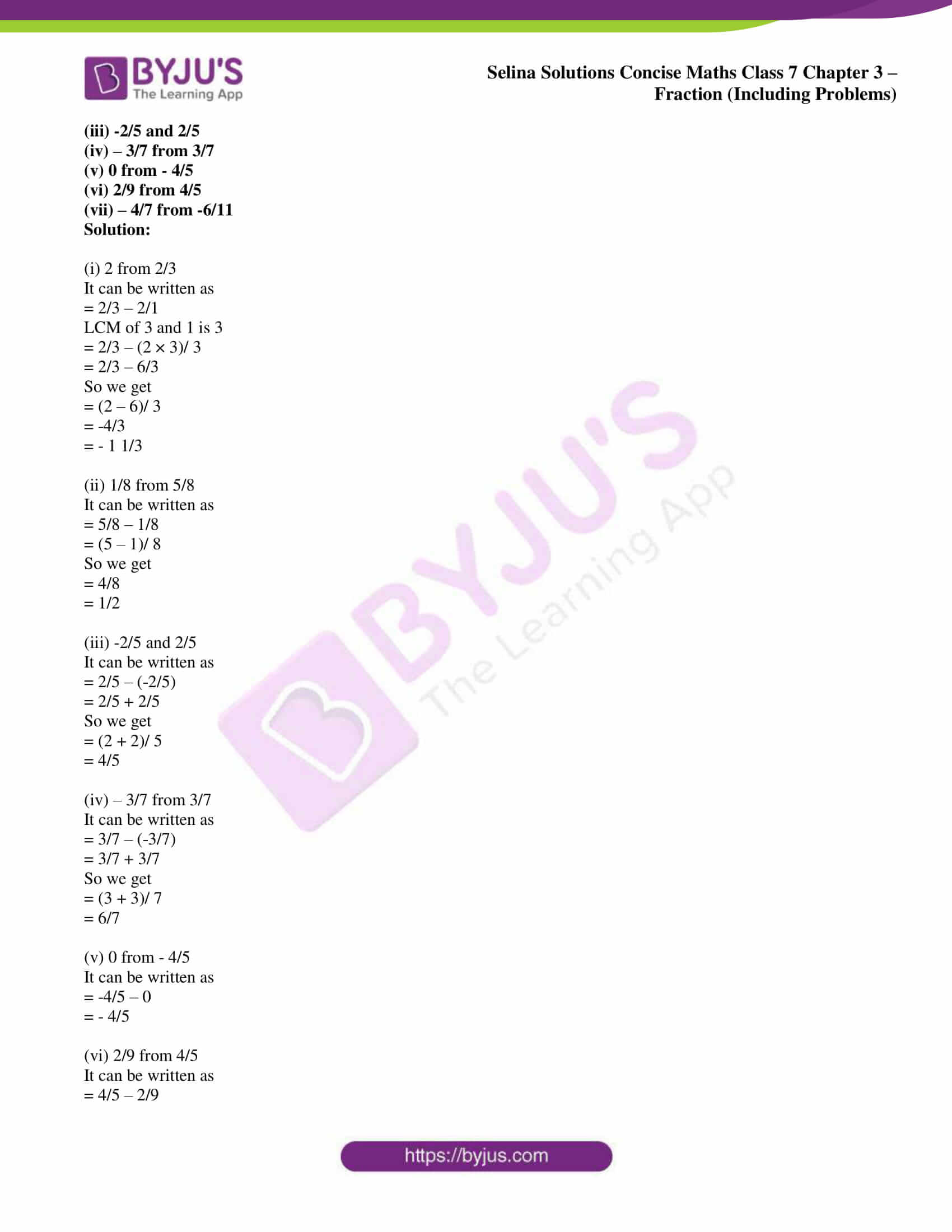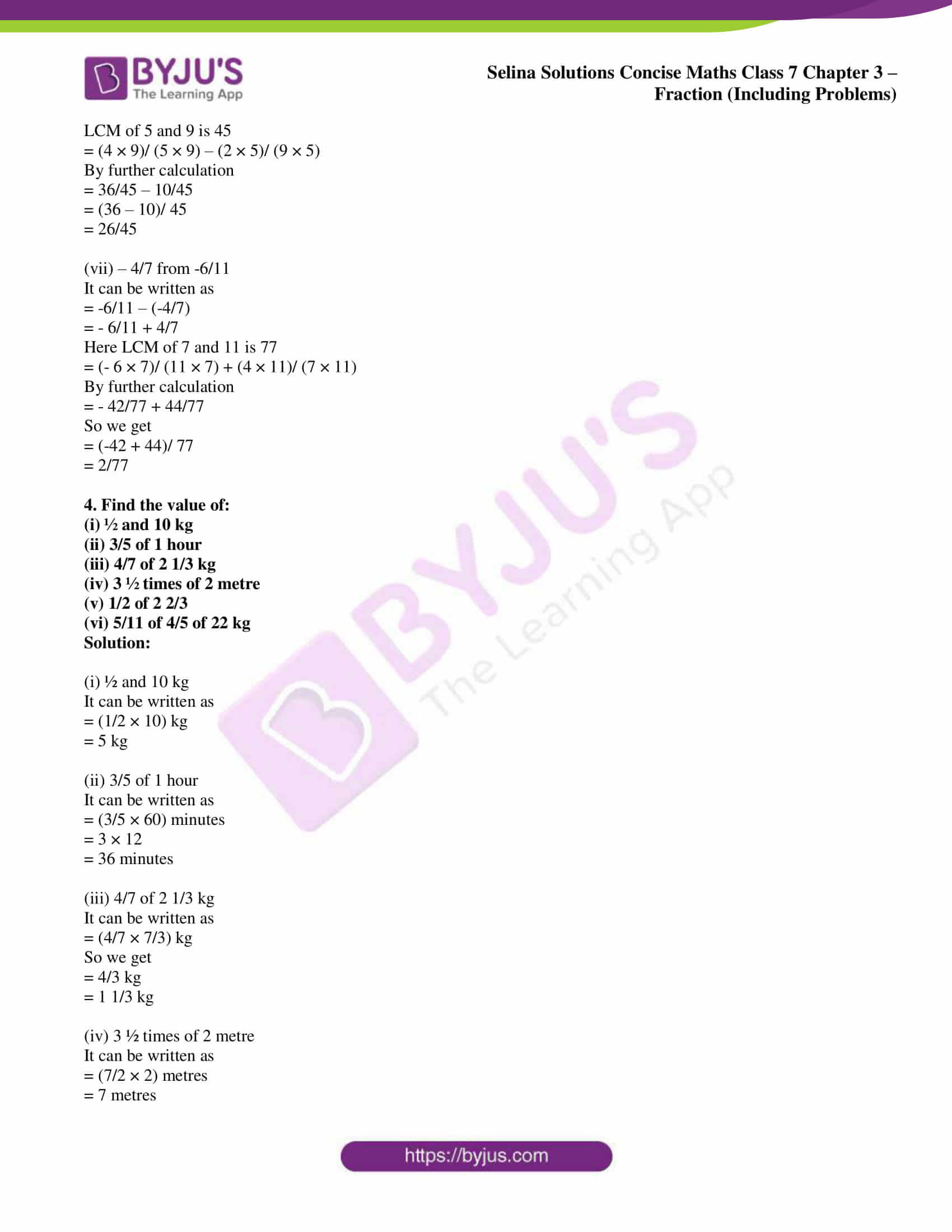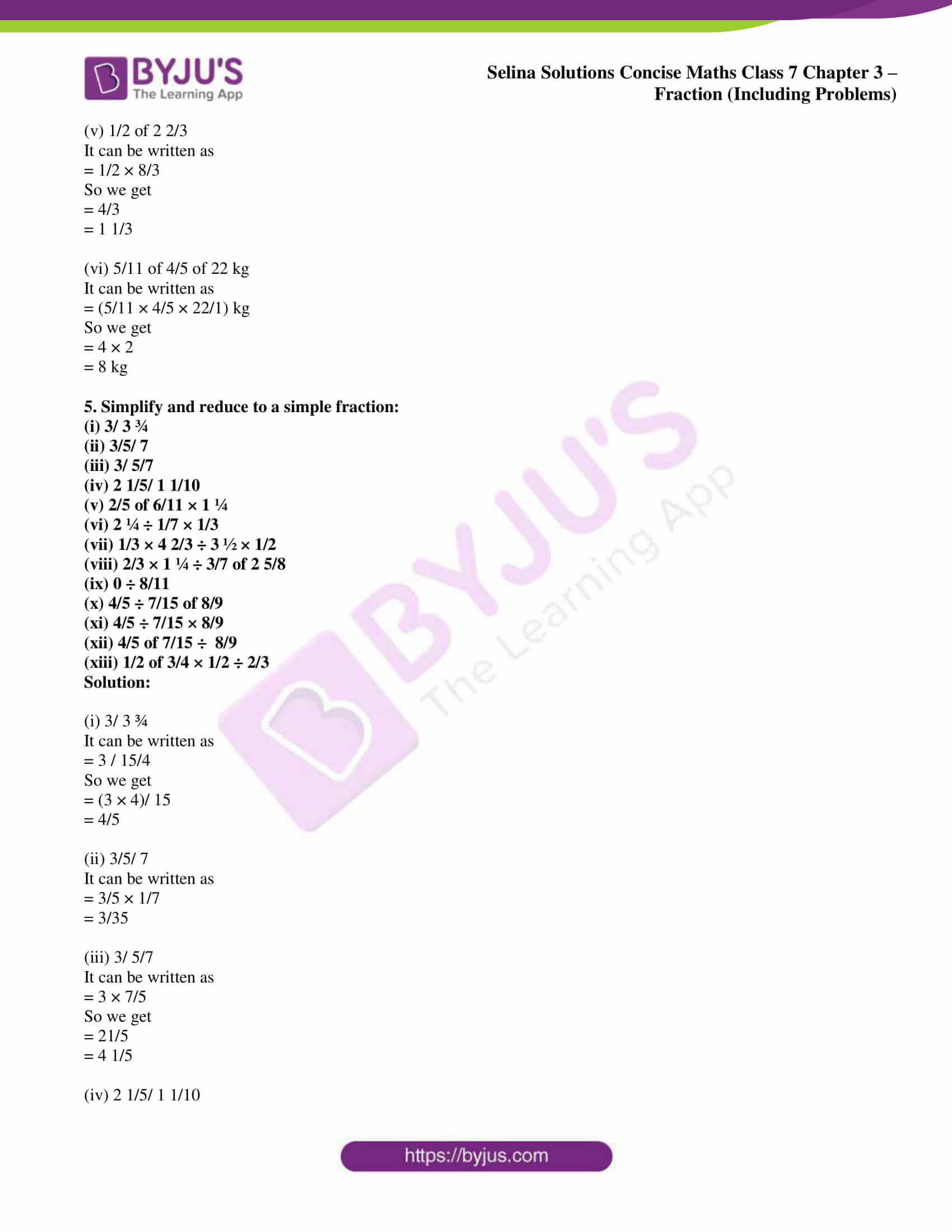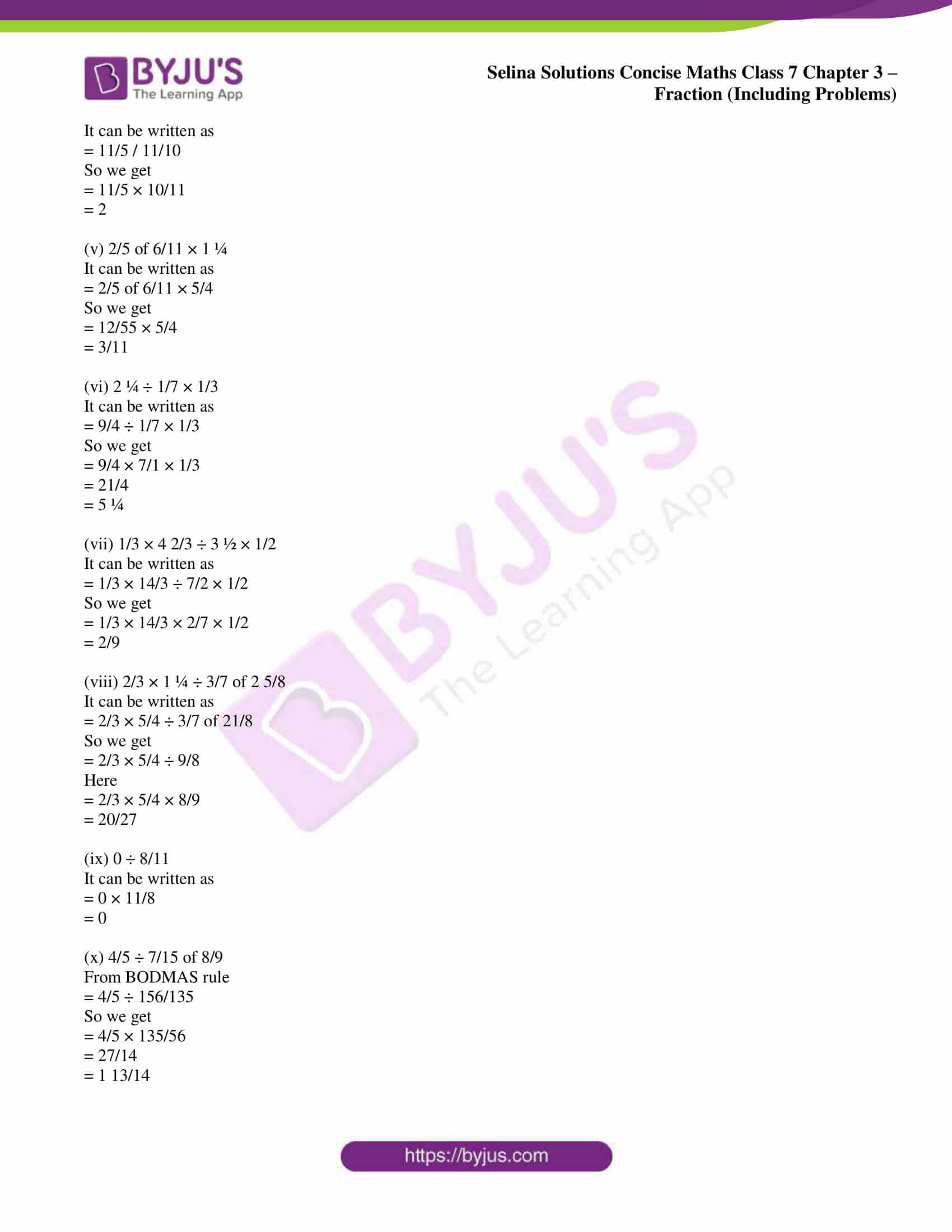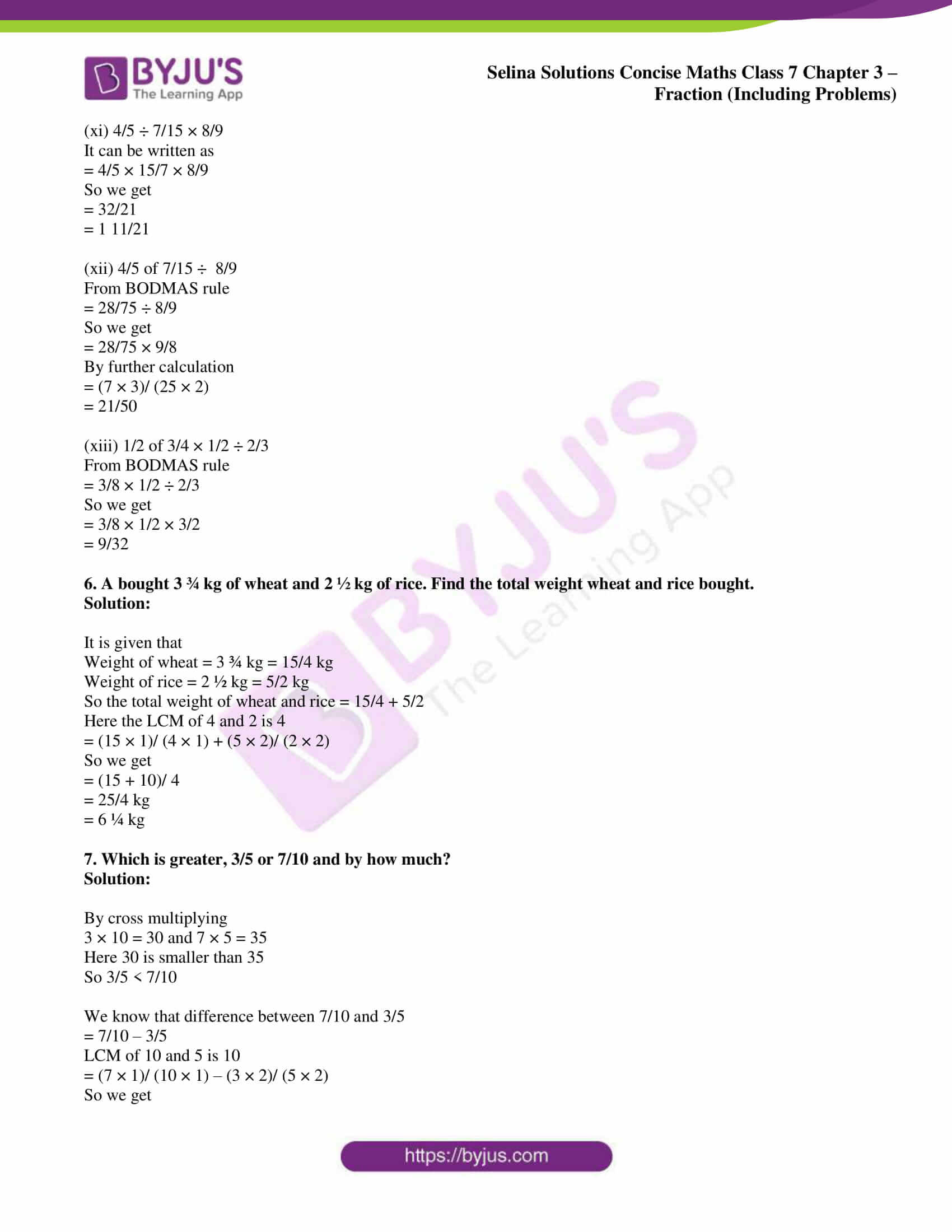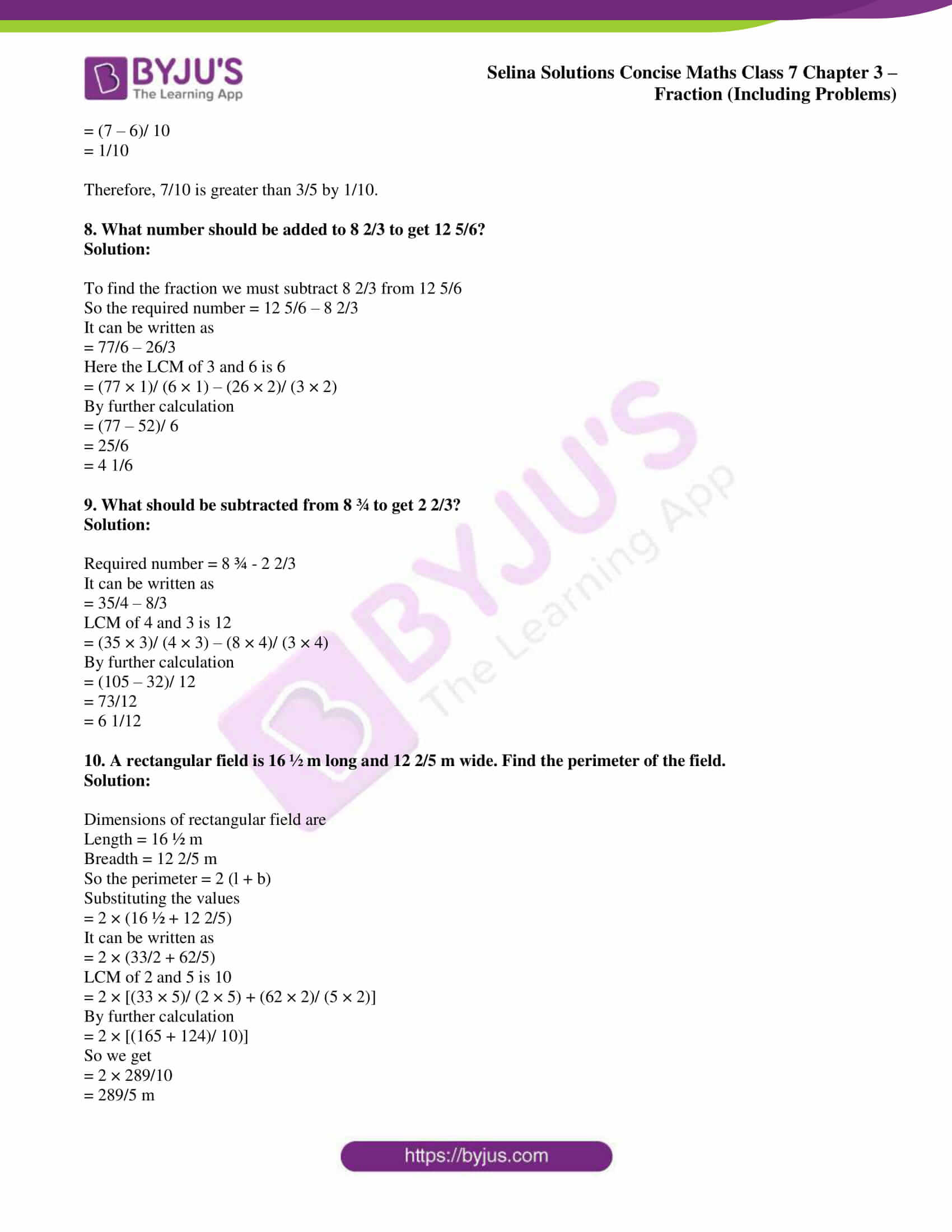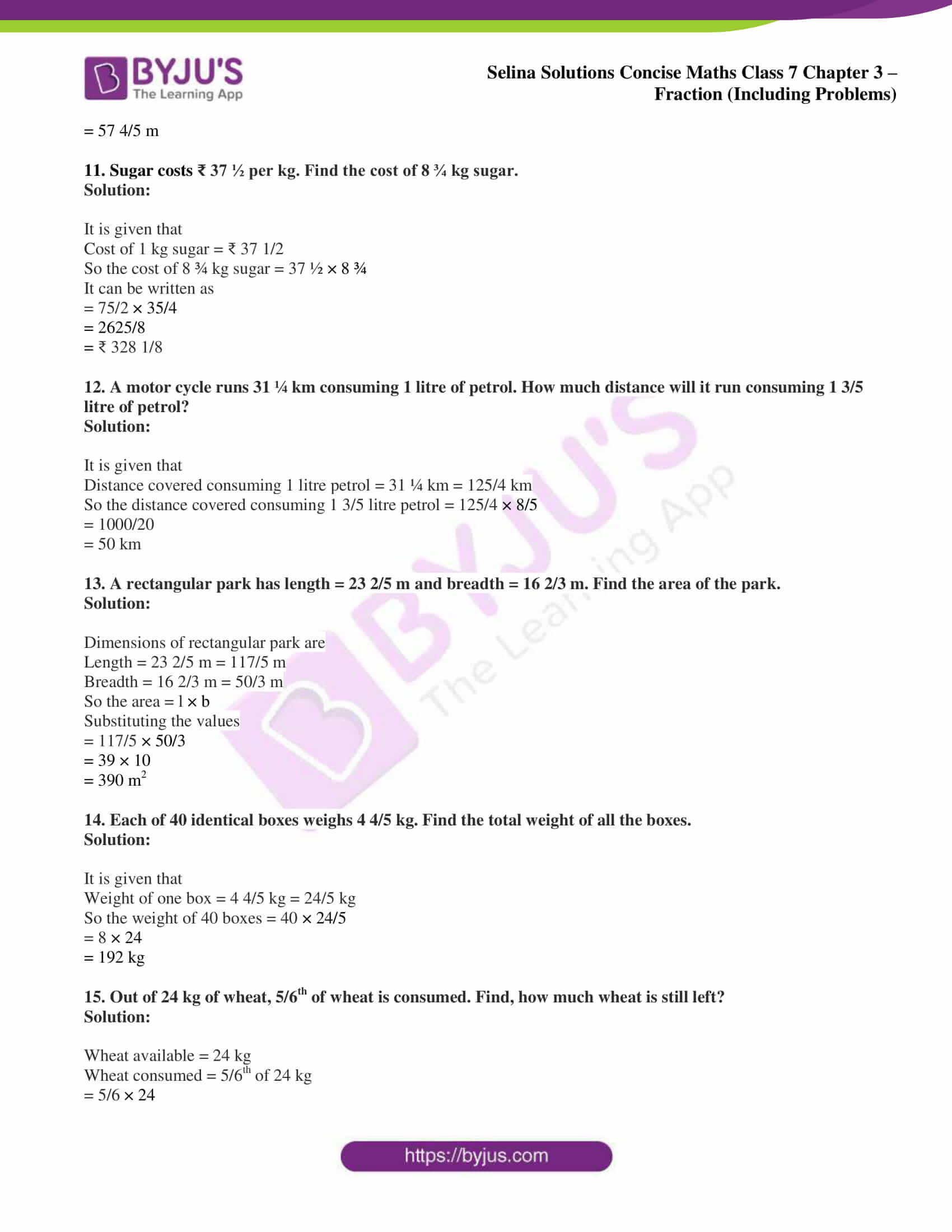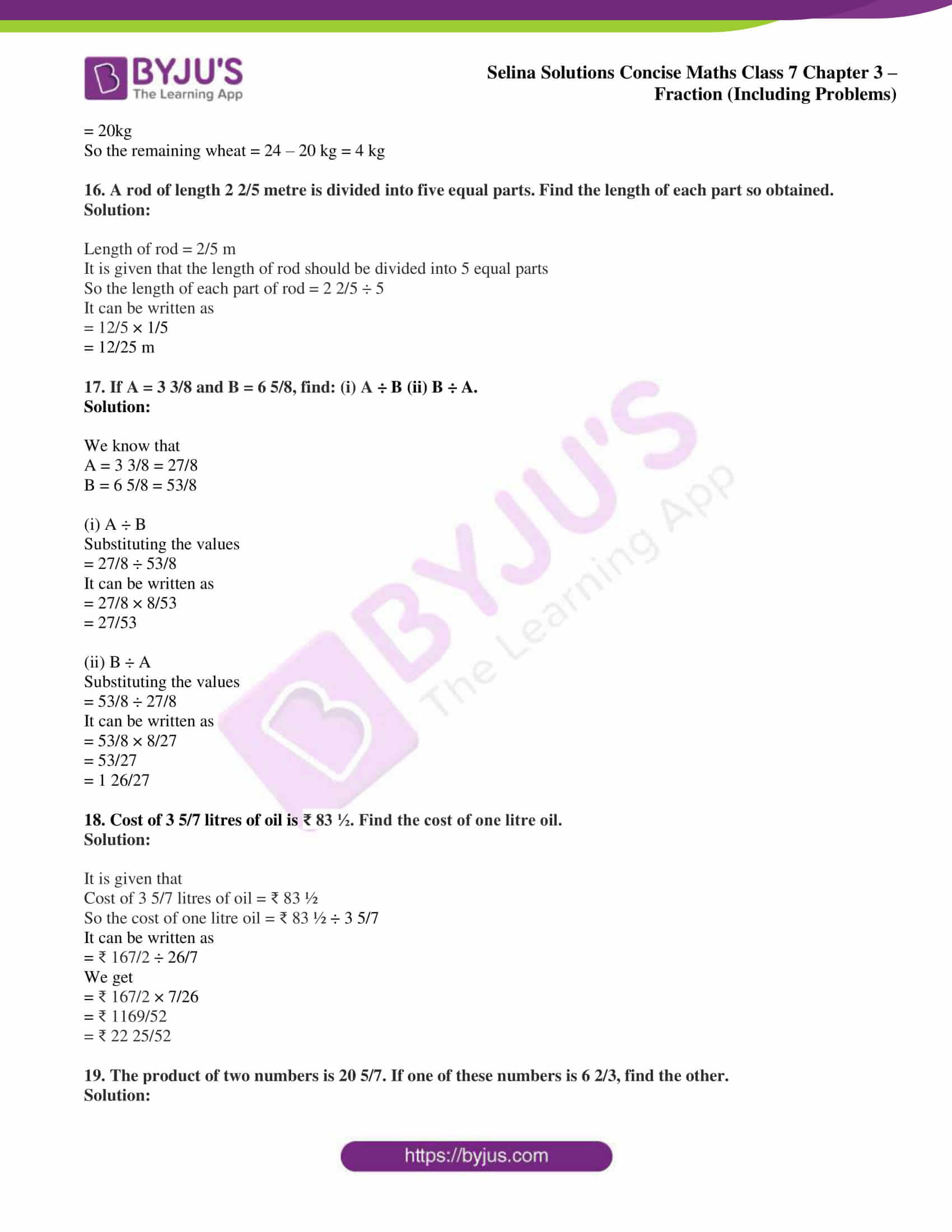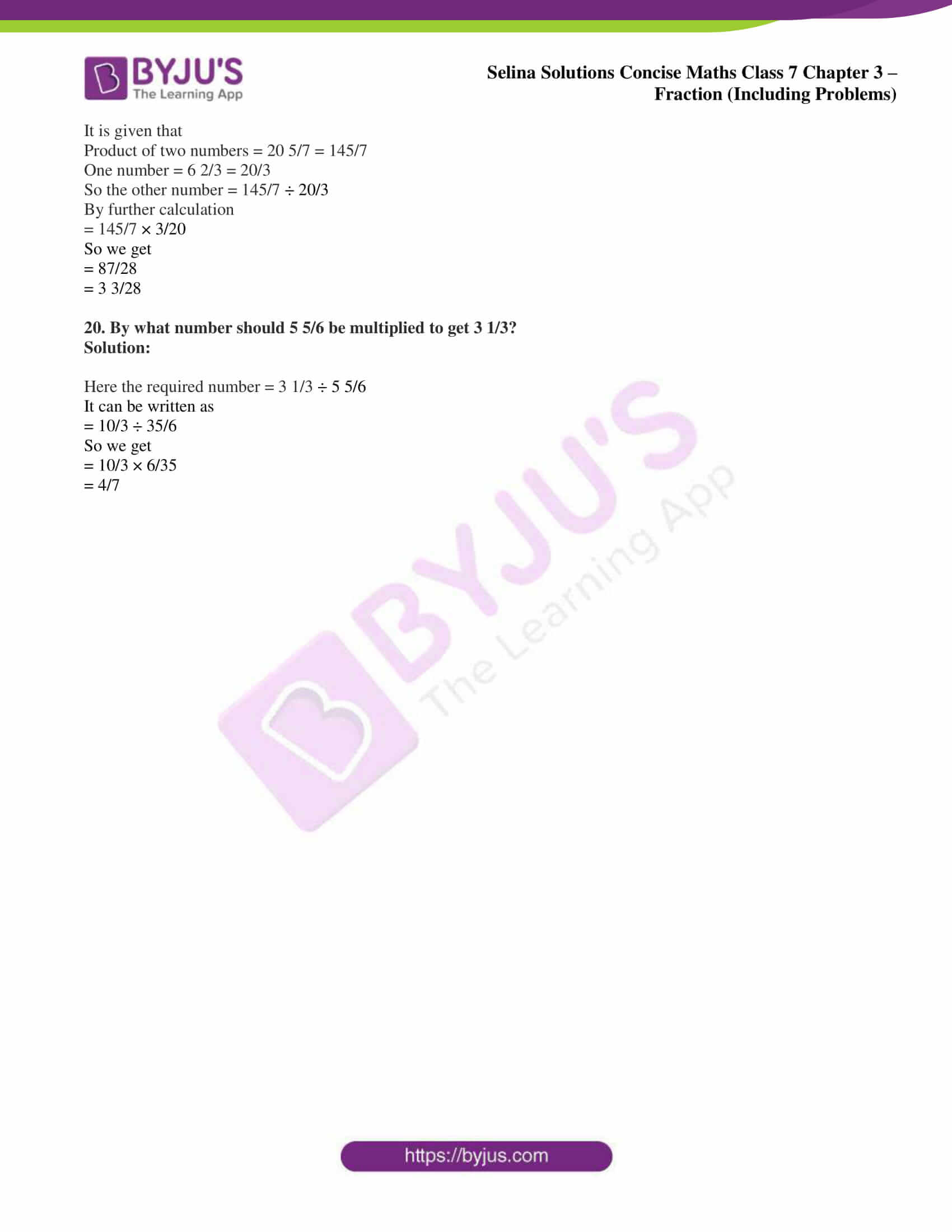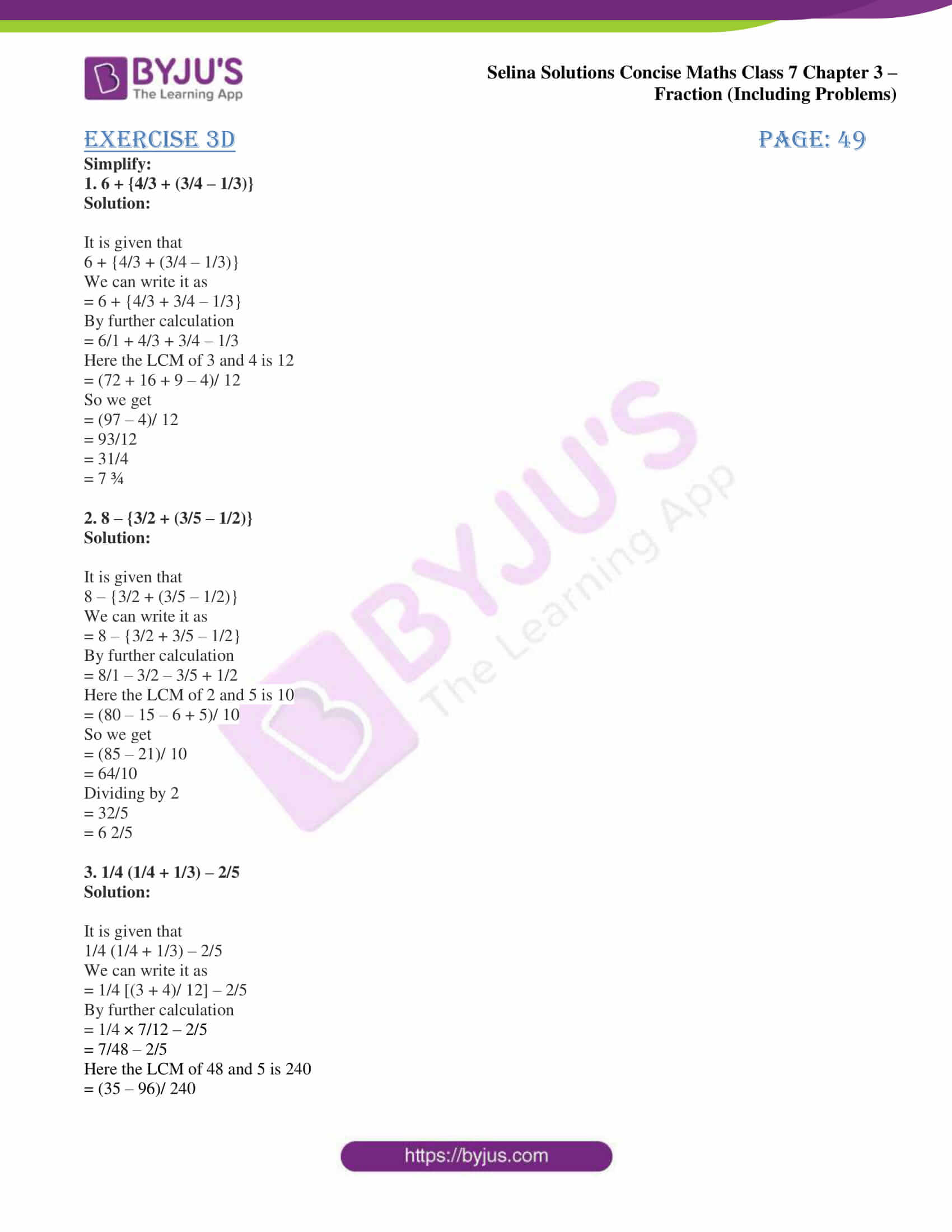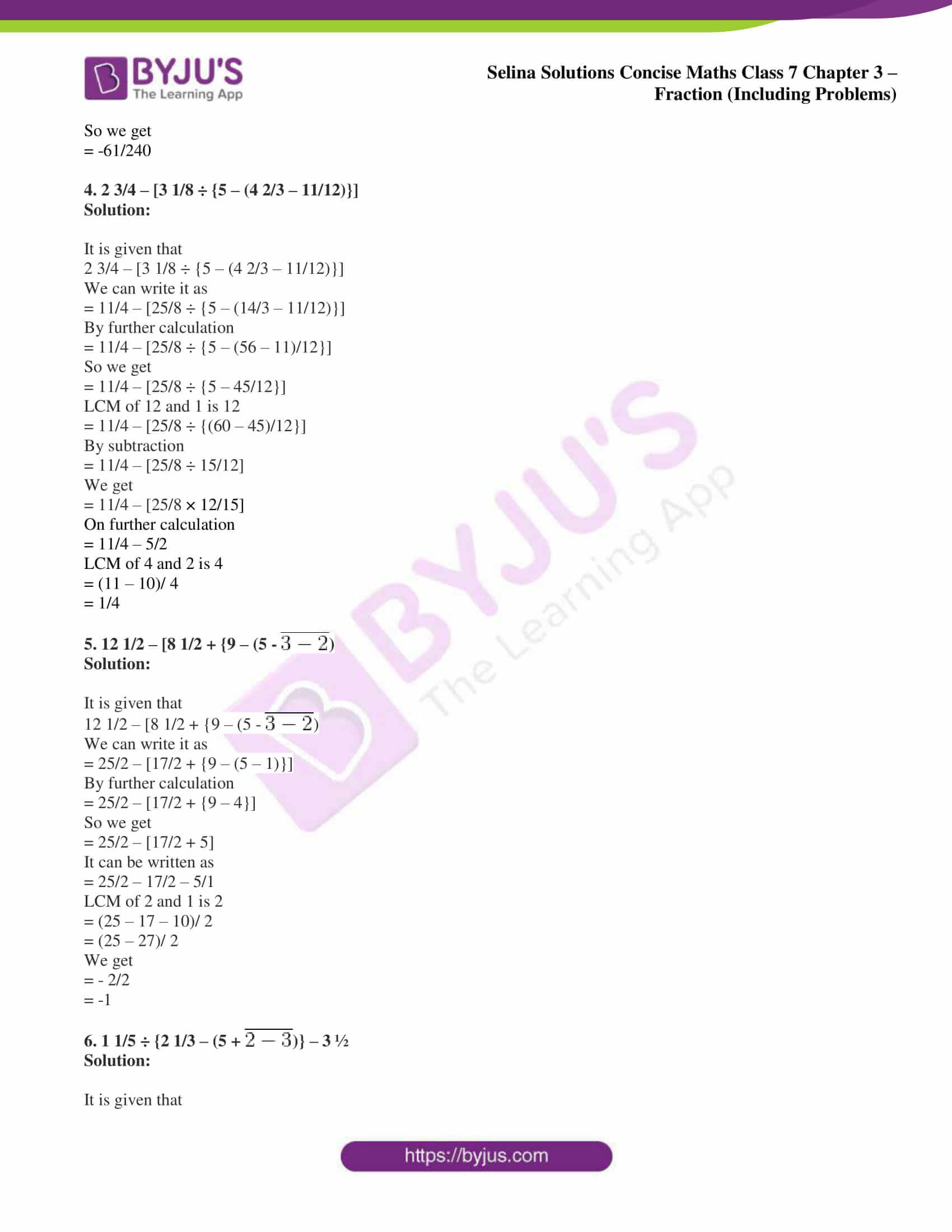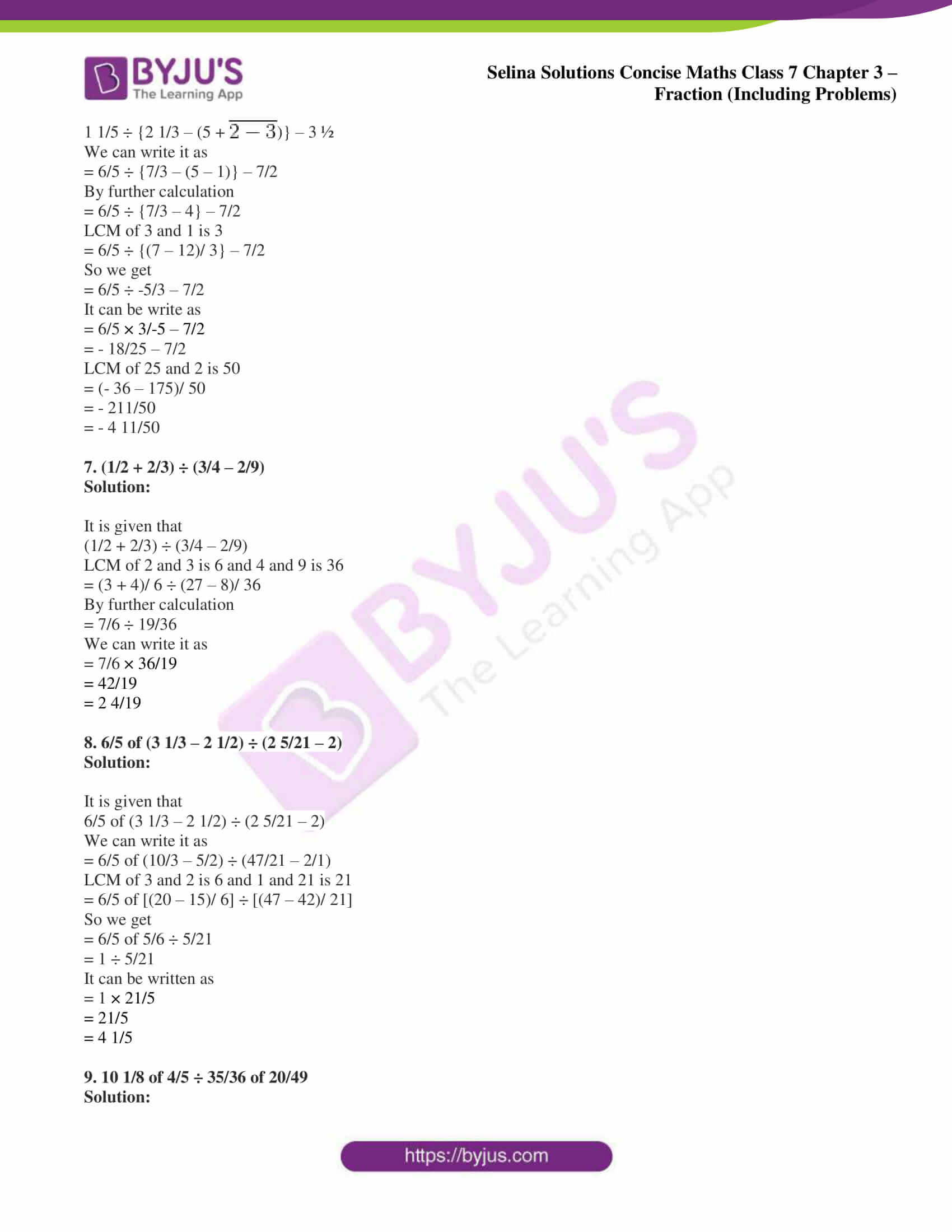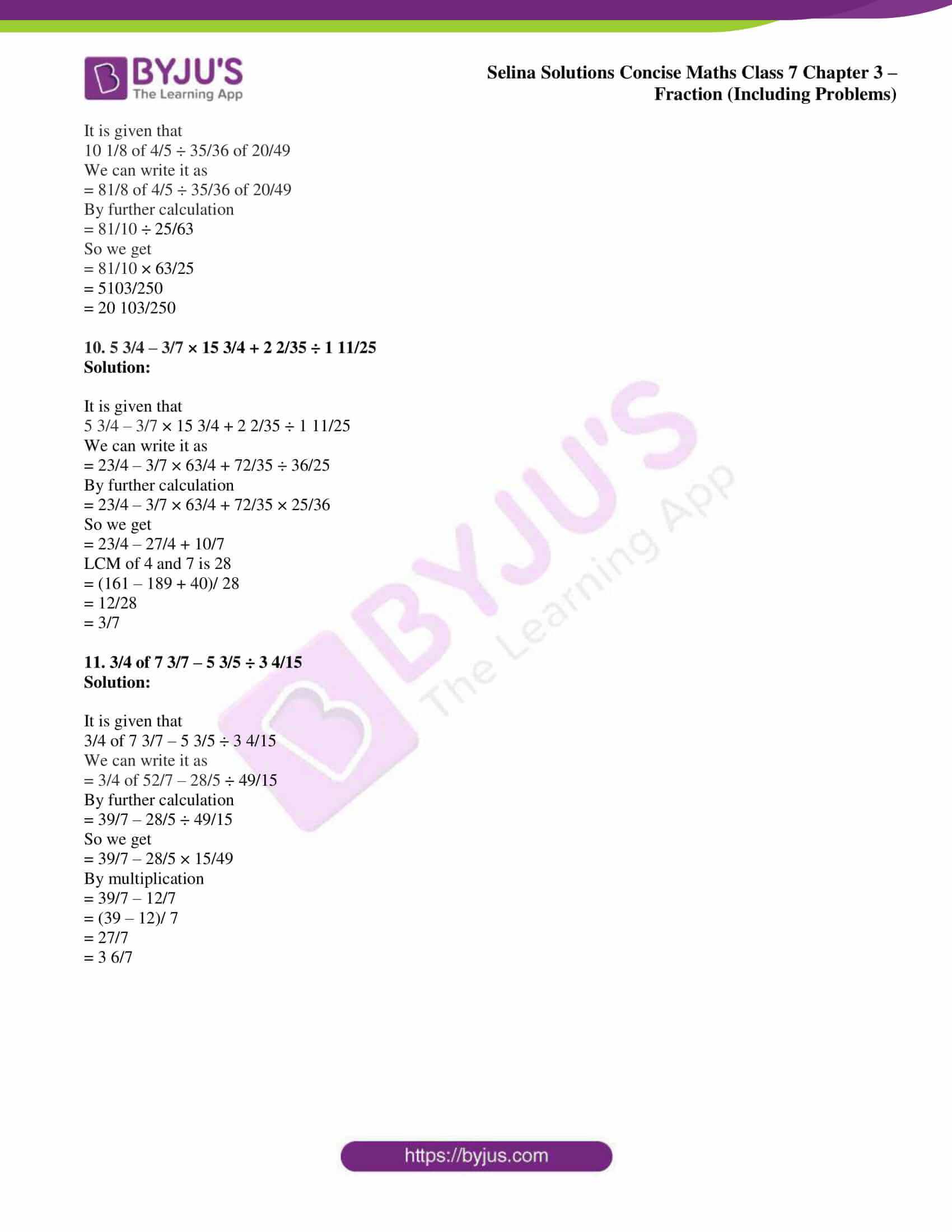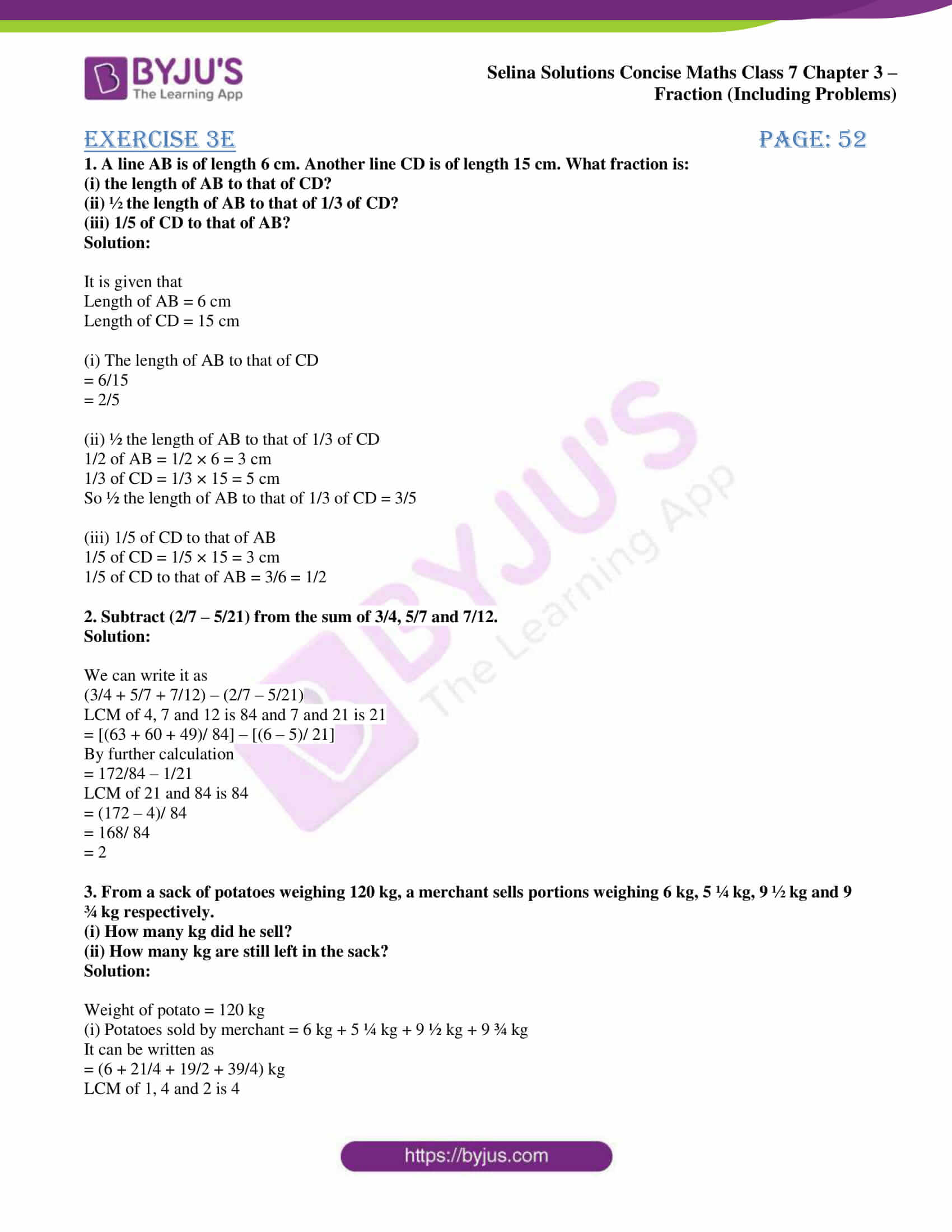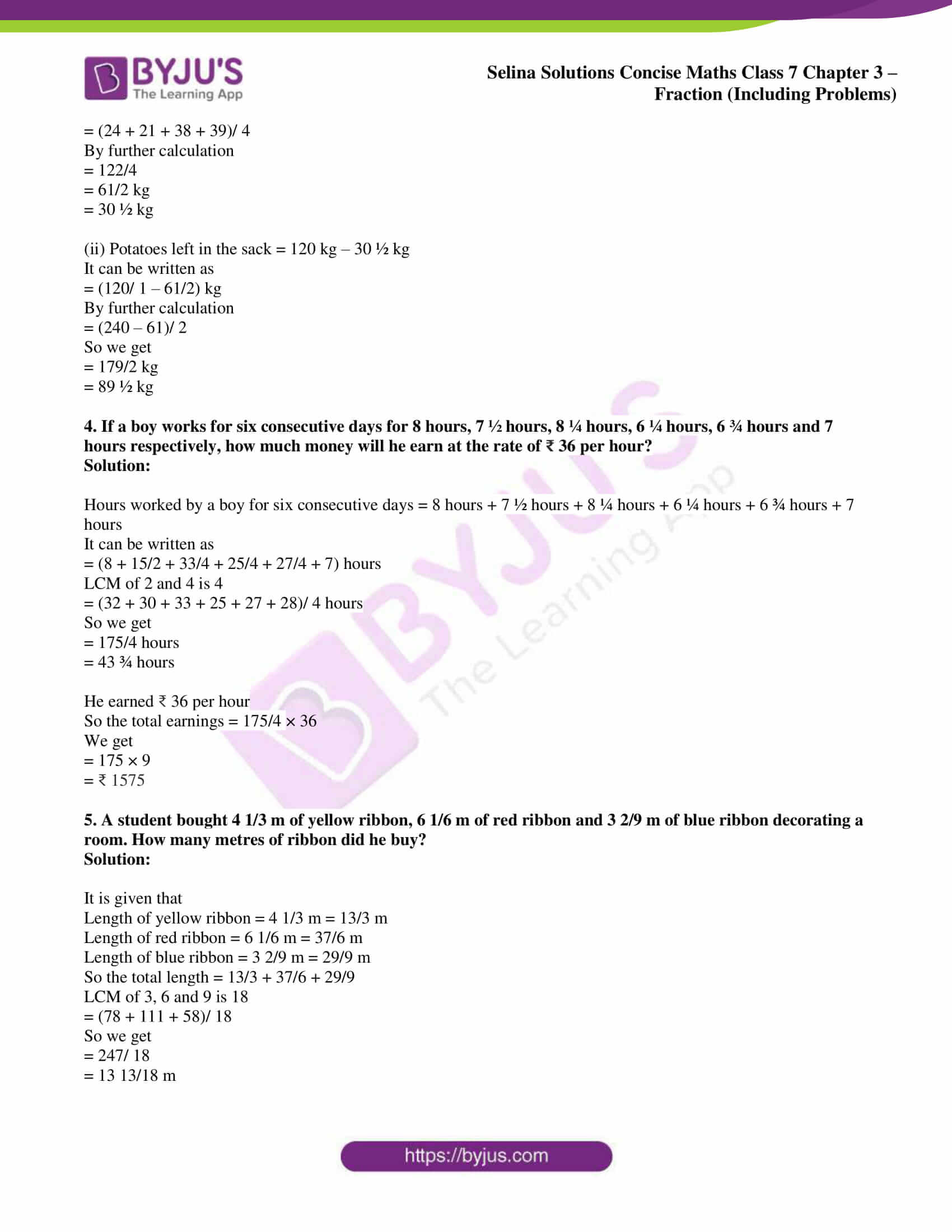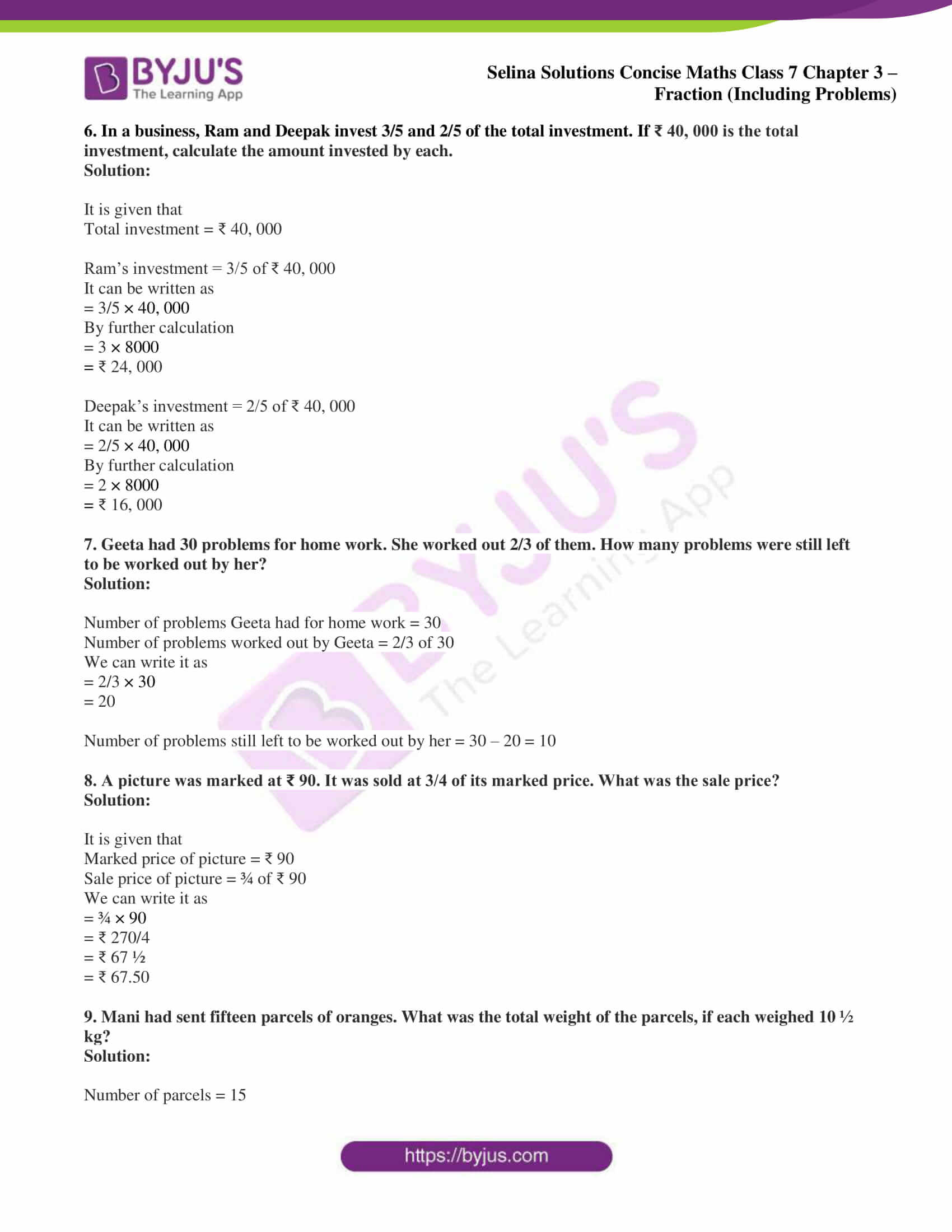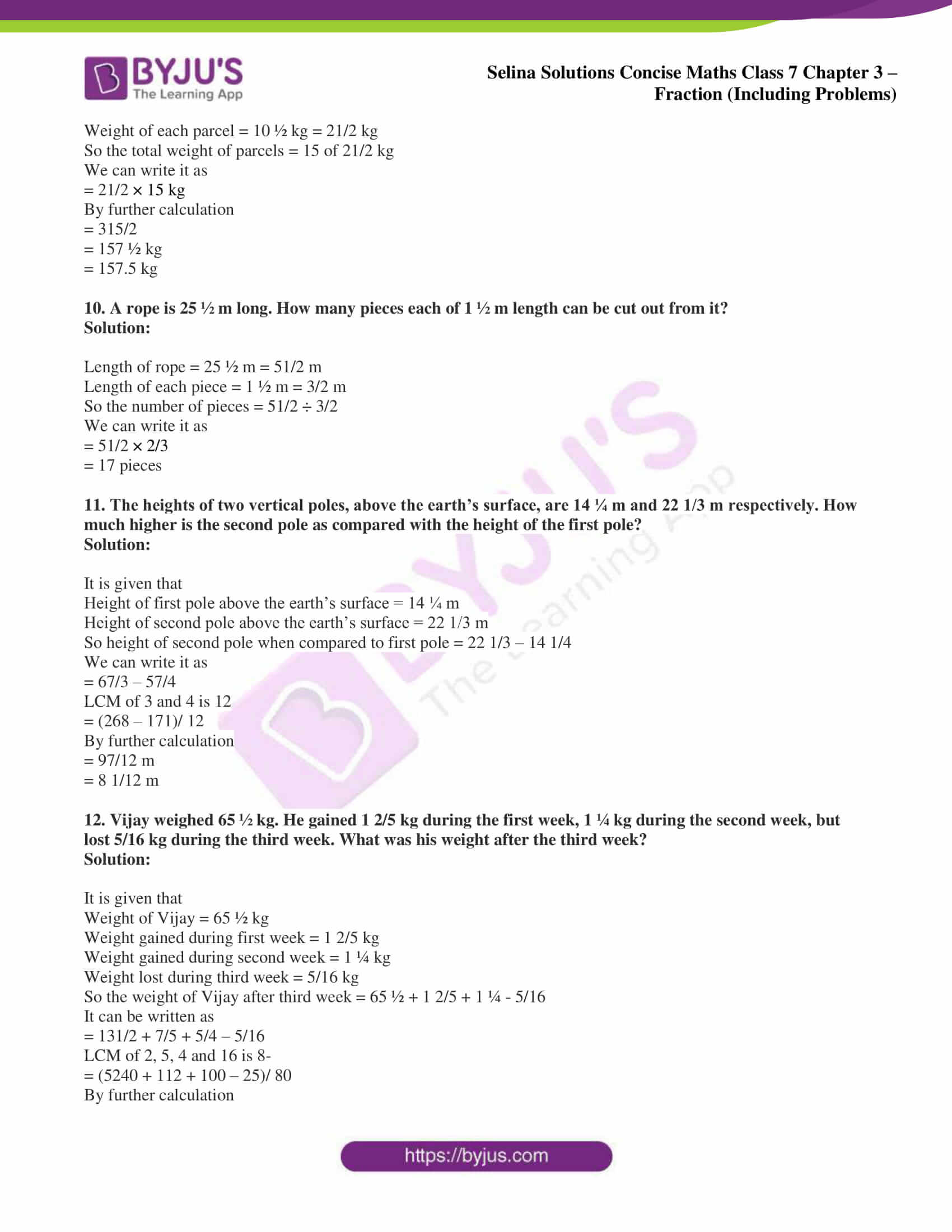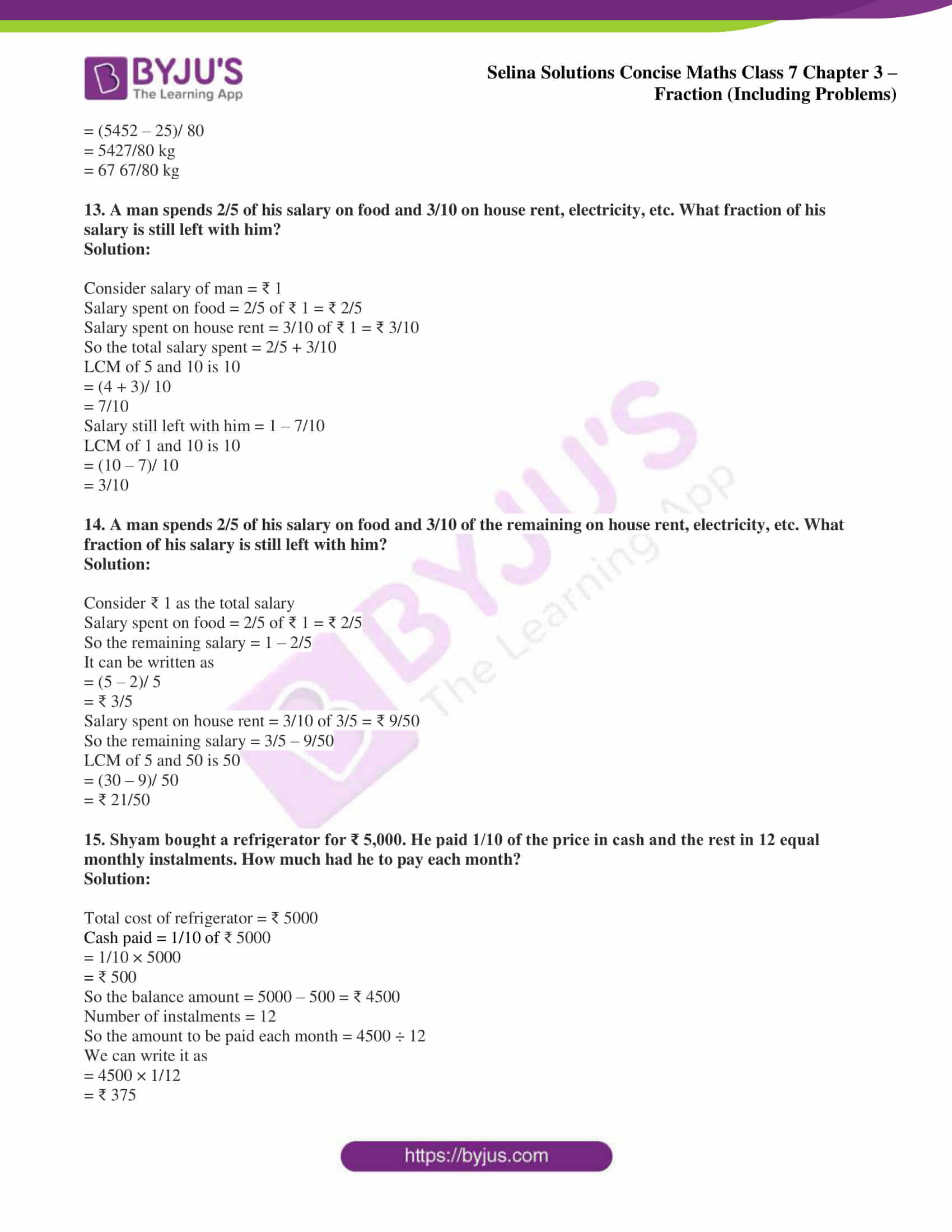## Access Selina Solutions Concise Maths Class 7 Chapter 3: Fraction (Including Problems)

#### Exercise 3A page: 39

1. Classify each fraction given below as decimal or vulgar fraction, proper or improper fraction and mixed fraction:

(i) 3/5

(ii) 11/10

(iii) 13/20

(iv) 18/7

(v) 3 2/9

Solution:

(i) 3/5 is a vulgar and proper fraction.

(ii) 11/10 is a decimal and improper fraction.

(iii) 13/20 is a decimal and proper fraction.

(iv) 18/7 is a vulgar and improper fraction.

(v) 3 2/9 is a mixed fraction.

2. Express the following improper fractions as mixed fractions:

(i) 18/5

(ii) 7/4

(iii) 25/6

(iv) 38/5

(v) 22/5

Solution:

(i) 18/5 can be expressed as mixed fractions as 3 3/5.

(ii) 7/4 can be expressed as mixed fractions as 1 3/4.

(iii) 25/6 can be expressed as mixed fractions as 4 1/6.

(iv) 38/5 can be expressed as mixed fractions as 7 3/5.

(v) 22/5 can be expressed as mixed fractions as 4 2/5.

3. Express the following mixed fractions as improper fractions:

(i) 2 4/9

(ii) 7 5/13

(iii) 3 1/4

(iv) 2 5/48

(v) 12 7/11

Solution:

(i) 2 4/9

It can be written as

= (2 × 9 + 4)/ 9

By further calculation

= (18 + 4)/ 9

= 22/9

(ii) 7 5/13

It can be written as

= (7 × 13 + 5)/ 13

By further calculation

= (91 + 5)/ 13

= 96/13

(iii) 3 1/4

It can be written as

= (3 × 4 + 1)/ 4

By further calculation

= (12 + 1)/ 4

= 13/4

(iv) 2 5/48

It can be written as

= (2 × 48 + 5)/ 48

By further calculation

= (96 + 5)/ 48

= 101/48

(v) 12 7/11

It can be written as

= (12 × 11 + 7)/ 11

By further calculation

= (132 + 7)/ 11

= 139/11

4. Reduce the given fractions to lowest terms:

(i) 8/18

(ii) 27/36

(iii) 18/42

(iv) 35/75

(v) 18/45

Solution:

(i) 8/18

Here the HCF of 8 and 18 is 2

So by dividing numerator and denominator by 2

= (8 ÷ 2)/ (18 ÷ 2)

We get

= 4/9

(ii) 27/36

Here the HCF of 27 and 36 is 9

So by dividing numerator and denominator by 9

= (27 ÷ 9)/ (36 ÷ 9)

We get

= 3/4

(iii) 18/42

Here the HCF of 18 and 42 is 6

So by dividing both numerator and denominator by 6

= (18 ÷ 6)/ (42 ÷ 6)

We get

= 3/7

(iv) 35/75

Here the HCF of 35 and 75 is 5

So by dividing both numerator and denominator by 5

= (35 ÷ 5)/ (75 ÷ 5)

We get

= 7/15

(v) 18/45

Here the HCF of 18 and 45 is 9

So by dividing both numerator and denominator by 9

= (18 ÷ 9)/ (45 ÷ 9)

We get

= 2/5

5. State true or false:

(i) 30/40 and 12/16 are equivalent fractions.

(ii) 10/25 and 25/10 are equivalent fractions.

(iii) 35/49, 20/28, 45/63 and 100/140 are equivalent fractions.

Solution:

(i) True.

Here 30/40 = 3/4 and 12/16 = 3/4

(ii) False.

Here 10/25 = 2/5 and 25/10 = 5/2

(iii) True.

35/49 = 5/7, 20/28 = 5/7, 45/63 = 5/7 and 100/140 = 5/7 where all are equal.

6. Distinguish each of the fractions, given below, as a simple fraction or a complex fraction:

(i) 0/8

(ii) -3/-8

(iii) 5/-7

(iv) 3 3/5/ 18

(v) -6/ 2 2/5

(vi) 3 1/3/ 7 2/7

(vii) – 5 2/9/ 5

(viii) -8/0

Solution:

(i) 0/8 is a simple fraction.

(ii) -3/-8 is a simple fraction.

(iii) 5/-7 is a simple fraction.

(iv) 3 3/5/ 18 is a complex fraction.

(v) -6/ 2 2/5 is a complex fraction.

(vi) 3 1/3/ 7 2/7 is a complex fraction.

(vii) – 5 2/9/ 5 is a complex fraction.

(viii) -8/0 is neither a complex nor a simple fraction.

#### Exercise 3B page: 43

1. For each pair, given below, state whether it forms like fractions or unlike fractions:

(i) 5/8 and 7/8

(ii) 8/15 and 8/21

(iii) 4/9 and 9/4

Solution:

(i) 5/8 and 7/8 are like fractions.

(ii) 8/15 and 8/21 are unlike fractions.

(iii) 4/9 and 9/4 are unlike fractions.

2. Convert given fractions into fractions with equal denominators:

(i) 5/6 and 7/9

(ii) 2/3, 5/6 and 7/12

(iii) 4/5, 17/20, 23/20 and 11/16

Solution:

(i) 5/6 and 7/9

Here the LCM of 6 and 9 is 18

5/6 = (5 × 3)/ (6 × 3) = 15/18

7/9 = (7 × 2)/ (9 × 2) = 14/18

Therefore, 15/18 and 14/18 are the required fractions.

(ii) 2/3, 5/6 and 7/12

Here the LCM of 3, 6 and 12 is 12

2/3 = (2 × 4)/ (3 × 4) = 8/12

5/6 = (5 × 2)/ (6 × 2) = 10/12

7/12 = 7/12

Therefore, 8/12, 10/12 and 7/12 are the required fractions.

(iii) 4/5, 17/20, 23/40 and 11/16

Here the LCM of 5, 20, 40 and 16 is 80

4/5 = (4 × 16)/ (5 × 16) = 64/80

17/20 = (17 × 4)/ (20 × 4) = 68/80

23/40 = (23 × 2)/ (40 × 2) = 46/80

11/16 = (11 × 5)/ (16 × 5) = 55/80

Therefore, 64/80, 68/80, 46/80 and 55/80 are the required fractions.

3. Convert given fractions into fractions with equal numerators:

(i) 8/9 and 12/17

(ii) 6/13, 15/23 and 12/17

(iii) 15/19, 25/28, 9/11 and 45/47

Solution:

(i) 8/9 and 12/17

Here the LCM of 8 and 12 is 24

8/9 = (8 × 3)/ (9 × 3) = 24/27

12/17 = (12 × 2)/ (17 × 2) = 24/34

Therfore, 24/27 and 24/34 are the required fractions.

(ii) 6/13, 15/23 and 12/17

Here the LCM of 6, 15 and 12 is 60

6/13 = (6 × 10)/ (13 × 10) = 60/130

15/23 = (15 × 4)/ (23 × 4) = 60/92

12/17 = (12 × 5)/ (17 × 5) = 60/85

Therefore, 60/130, 60/92 and 60/85 are the required fractions.

(iii) 15/19, 25/28, 9/11 and 45/47

Here the LCM of 15, 25, 9 and 45 is 225

15/19 = (15 × 15)/ (19 × 15) = 225/285

25/28 = (25 × 9)/ (28 × 9) = 225/252

9/11 = (9 × 25)/ (11 × 25) = 225/275

45/47 = (45 × 5)/ (47 × 5) = 225/ 235

Therefore, 225/285, 225/252, 225/275 and 225/235 are the required fractions.

4. Put the given fractions in ascending order by making denominators equal:

(i) 1/3, 2/5, 3/4 and 1/6

(ii) 5/6, 7/8, 11/12 and 3/10

(iii) 5/7, 3/8, 9/14 and 20/21

Solution:

(i) 1/3, 2/5, 3/4 and 1/6

Here the LCM of 3, 5, 4 and 6 is 60

1/3 = (1 × 20)/ (3 × 20) = 20/60

2/5 = (2 × 12)/ (5 × 12) = 24/60

3/4 = (3 × 15)/ (4 × 15) = 45/60

1/6 = (1 × 10)/ (6 × 10) = 10/60

So we get

10/60 < 20/60 < 24/60 < 45/60

It can be written as

1/6 < 1/3 < 2/5 < 3/4

Therefore, 1/6, 1/3, 2/5 and 3/4 are in ascending order.

(ii) 5/6, 7/8, 11/12 and 3/10

Here the LCM of 6, 8, 12 and 10 is 240

5/6 = (5 × 40)/ (6 × 40) = 200/240

7/8 = (7 × 30)/ (8 × 30) = 210/240

11/12 = (11 × 20)/ (12 × 20) = 220/240

3/10 = (3 × 24)/ (10 × 24) = 72/240

So we get

72/240 < 200/240 < 210/240 < 220/240

It can be written as

3/10 < 5/6 < 7/8 < 11/12

Therefore, 3/10, 5/6, 7/8 and 11/12 are in ascending order.

(iii) 5/7, 3/8, 9/14 and 20/21

Here the LCM of 7, 8, 14 and 21 is 168

5/7 = (5 × 24)/ (7 × 24) = 120/168

3/8 = (3 × 21)/ (8 × 21) = 63/168

9/14 = (9 × 12)/ (14 × 12) = 108/168

20/21 = (20 × 8)/ (21 × 8) = 160/168

So we get

63/168 < 108/ 168 < 120/168 < 160/168

It can be written as

3/8 < 9/14 < 5/7 < 20/21

Therefore, 3/8, 9/14, 5/7 and 20/21 are in ascending order.

5. Arrange the given fractions in descending order by making numerators equal:

(i) 5/6, 4/15, 8/9 and 1/3

(ii) 3/7, 4/9, 5/7 and 8/11

(iii) 1/10, 6/11, 8/11 and 3/5

Solution:

(i) 5/6, 4/15, 8/9 and 1/3

Here the LCM of 5, 4, 8 and 1 is 40

5/6 = (5 × 8)/ (6 × 8) = 40/48

4/15 = (4 × 10)/ (15 × 10) = 40/150

8/9 = (8 × 5)/ (9 × 5) = 40/45

1/3 = (1 × 40)/ (3 × 40) = 40/120

So we get

40/45 > 40/48 > 40/120 > 40/150

It can be written as

8/9 > 5/6 > 1/3 > 4/15

Therefore, 8/9, 5/6, 1/3 and 4/15 are in descending order.

(ii) 3/7, 4/9, 5/7 and 8/11

Here the LCM of 3, 4, 5 and 8 is 120

3/7 = (3 × 40)/ (7 × 40) = 120/280

4/9 = (4 × 30)/ (9 × 30) = 120/270

5/7 = (5 × 24)/ (7 × 24) = 120/168

8/11 = (8 × 15)/ (11 × 15) = 120/165

So we get

120/165 > 120/168 > 120/270 > 120/280

It can be written as

8/11 > 5/7 > 4/9 > 3/7

Therefore, 8/11, 5/7, 4/9 and 3/7 are in descending order.

(iii) 1/10, 6/11, 8/11 and 3/5

Here the LCM of 1, 6, 8 and 3 is 24

1/10 = (1 × 24)/ (10 × 24) = 24/240

6/11 = (6 × 4)/ (11 × 4) = 24/44

8/11 = (8 × 3)/ (11 × 3) = 24/33

3/5 = (3 × 8)/ (5 × 8) = 24/40

So we get

24/33 > 24/40 > 24/44 > 24/240

It can be written as

8/11 > 3/5 > 6/11 > 1/10

Therefore, 8/11, 3/5, 6/11 and 1/10 are in descending order.

6. Find the greater fraction:

(i) 3/5 and 11/15

(ii) 4/5 and 3/10

(iii) 6/7 and 5/9

Solution:

(i) 3/5 and 11/15

Here the LCM of 5 and 15 is 15

3/5 = (3 × 3)/ (5 × 3) = 9/ 15

11/15 = 11/15

So we get, 11/15 > 9/15

Therefore, 11/15 is greater.

(ii) 4/5 and 3/10

Here the LCM of 5 and 10 is 10

4/5 = (4 × 2)/ (5 × 2) = 8/10

3/10 = 3/10

So we get, 8/10 > 3/10

4/5 > 3/10

Therefore, 4/5 is greater.

(iii) 6/7 and 5/9

Here LCM of 7 and 9 is 63

6/7 = (6 × 9)/ (7 × 9) = 54/63

5/9 = (5 × 7)/ (9 × 7) = 35/63

So we get, 54/63 > 35/63

6/7 > 35/63

Therefore, 6/7 is greater.

7. Insert one fraction between:
(i) 3/7 and 4/9

(ii) 2 and 8/3

(iii) 9/17 and 6/13

Solution:

(i) 3/7 and 4/9

So the fraction between 3/7 and 4/9

= (3 + 4)/ (7 + 9)

= 7/16

(ii) 2 and 8/3

So the fraction between 2 and 8/3

= (2 + 8)/ (1 + 3)

= 10/4

Dividing by 2

= 5/2

= 2 ½

(iii) 9/17 and 6/13

So the fraction between 9/17 and 6/13

= (9 + 6)/ (17 + 13)

= 15/30

By division

= 1/2

8. Insert three fractions between:

(i) 2/5 and 4/9

(ii) 1/2 and 5/7

(iii) 3/8 and 6/11

Solution:

(i) 2/5 and 4/9

So the fraction between 2/5 and 4/9

= (2 + 4)/ (5 + 9)

= 6/14

Dividing by 2

= 3/7

Fraction between 2/5 and 3/7

= (2 + 3)/ (5 + 7)

= 5/12

Fraction between 3/7 and 4/9

= (3 + 4)/ (7 + 9)

= 7/16

Therefore, three fractions between 2/5 and 4/9 will be 5/12, 3/7 and 7/16.

(ii) 1/2 and 5/7

So the fraction between 1/2 and 5/7

= (1 + 5)/ (2 + 7)

= 6/ 9

Dividing by 3

= 2/ 3

Fraction between 1/2 and 2/3

= (1 + 2)/ (2 + 3)

= 3/ 5

Fraction between 2/3 and 5/7

= (2 + 5)/ (3 + 7)

= 7/ 10

Therefore, three fractions between 1/2 and 5/7 will be 3/5, 2/3 and 7/10.

(iii) 3/8 and 6/11

So the fraction between 3/8 and 6/11

= (3 + 6)/ (8 + 11)

= 9/ 19

Fraction between 3/8 and 9/19

= (3 + 9)/ (8 + 19)

= 12/27

= 4/9

Fraction between 9/19 and 6/11

= (9 + 6)/ (19 + 11)

= 15/30

= 1/2

Therefore, three fractions between 3/8 and 6/11 will be 4/9, 9/19 and 1/2.

9. Insert two fractions between:

(i) 1 and 3/11

(ii) 5/9 and 1/4

(iii) 5/6 and 1 1/5

Solution:

(i) 1 and 3/11

Fraction between 1 and 3/11

= (1 + 3)/ (1 + 11)

= 4/12

= 1/3

Fraction between 1/3 and 3/11

= (1 + 3)/ (3 + 11)

= 4/14

Dividing by 2

= 2/7

Therefore, two fractions between 1 and 3/11 will be 1/3 and 2/7.

(ii) 5/9 and 1/4

Fraction between 5/9 and 1/4

= (5 + 1)/ (9 + 4)

= 6/13

Fraction between 6/13 and 1/4

= (6 + 1)/ (13 + 4)

= 7/17

Therefore, two fractions between 5/9 and 1/4 will be 6/13 and 7/17.

(iii) 5/6 and 1 1/5

It can be written as

= 5/6 + 6/5

Fraction between 5/6 and 6/5

= (5 + 6)/ (6 + 5)

= 11/11

= 1

Fraction between 1 and 6/5

= (1 + 6)/ (1 + 5)

= 7/6

= 1 1/6

Therefore, two fractions between 5/6 and 1 1/5 will be 1 and 1 1/6.

#### Exercise 3C page: 46

1. Reduce to a single fraction:

(i) 1/2 + 2/3

(ii) 3/5 – 1/10

(iii) 2/3 – 1/6

(iv) 1 1/3 + 2 1/4

(v) 1/4 + 5/6 – 1/12

(vi) 2/3 – 3/5 + 3 – 1/5

(vii) 2/3 – 1/5 + 1/10

(viii) 2 1/2 + 2 1/3 – 1 1/4

(ix) 2 5/8 – 2 1/6 + 4 3/4

Solution:

(i) 1/2 + 2/3

Here the LCM of 2 and 3 is 6

= (1 × 3)/ (2 × 3) + (2 × 2)/ (3 × 2)

By further calculation

= 3/6 + 4/6

= (3 + 4)/ 6

So we get

= 7/6

= 1 1/6

(ii) 3/5 – 1/10

Here the LCM of 5 and 10 is 10

= (3 × 2)/ (5 × 2) – 1/10

By further calculation

= 6/10 – 1/10

So we get

= (6 – 1)/ 10

= 5/10

= 1/2

(iii) 2/3 – 1/6

Here the LCM of 3 and 6 is 6

= (2 × 2)/ (3 × 2) – 1/6

By further calculation

= 4/6 – 1/6

So we get

= (4 – 1)/ 6

= 3/6

= 1/2

(iv) 1 1/3 + 2 1/4

It can be written as

= 4/3 + 9/4

Here LCM of 3 and 4 is 12

= (4 × 4)/ (3 × 4) + (9 × 3)/ (4 × 3)

By further calculation

= 16/12 + 27/12

So we get

= (16 + 27)/ 12

= 43/12

= 3 7/12

(v) 1/4 + 5/6 – 1/12

Here LCM of 4, 6 and 12 is 12

= (1 × 3)/ (4 × 3) + (5 × 2)/ (6 × 2) – 1/12

By further calculation

= 3/12 + 10/12 – 1/12

So we get

= (3 + 10 – 1)/ 12

= 12/12

= 1

(vi) 2/3 – 3/5 + 3 – 1/5

Here LCM of 3 and 5 is 15

= (2 × 5)/ (3 × 5) – (3 × 3)/ (5 × 3) + (3 × 15)/ 15 – (1 × 3)/ (5 × 3)

By further calculation

= 10/15 – 9/15 + 45/15 – 3/15

So we get

= (10 – 9 + 45 – 3)/ 15

= (55 – 12)/ 15

= 43/15

= 2 13/15

(vii) 2/3 – 1/5 + 1/10

Here the LCM of 3, 5 and 10 is 30

= (2 × 10)/ (3 × 10) – (1 × 6)/ (5 × 6) + (1 × 3)/ (10 × 3)

By further calculation

= 20/30 – 6/30 + 3/30

So we get

= (20 – 6 + 3)/ 30

= (23 – 6)/ 30

= 17/30

(viii) 2 1/2 + 2 1/3 – 1 ¼

It can be written as

= 5/2 + 7/3 – 5/4

Here the LCM of 2, 3 and 4 is 12

= (5 × 6)/ (2 × 6) + (7 × 4)/ (3 × 4) – (5 × 3)/ (4 × 3)

By further calculation

= 30/12 + 28/12 – 15/12

So we get

= (30 + 28 – 15)/ 12

= (58 – 15)/ 12

= 43/12

= 3 7/12

(ix) 2 5/8 – 2 1/6 + 4 3/4

It can be written as

= 21/8 – 13/6 + 19/4

Here the LCM of 8, 6 and 4 is 24

= (21 × 3)/ (8 × 3) – (13 × 4)/ (6 × 4) + (19 × 6)/ (4 × 6)

By further calculation

= 63/24 – 52/24 + 114/24

So we get

= (63 – 52 + 114)/ 24

= (177 – 52)/ 24

= 125/24

= 5 5/24

2. Simplify:

(i) 3/4 × 6

(ii) 2/3 × 15

(iii) 3/4 × 1/2

(iv) 9/12 × 4/7

(v) 45 × 2 1/3

(vi) 36 × 3 1/4

(vii) 2 ÷ 1/3

(viii) 3 ÷ 2/5

(ix) 1 ÷ 3/5

(x) 1/3 ÷ 1/4

(xi) -5/8 ÷ 3/4

(xii) 3 3/7 ÷ 1 1/14

(xiii) 3 ¾ × 1 1/5 × 20/21

Solution:

(i) 3/4 × 6

It can be written as

= 3/4 × 6/1

= (3 × 6)/ (4 × 1)

So we get

= 18/4

HCF of 18 and 4 is 2

Dividing both numerator and denominator by 2

= (18 ÷ 2)/ (4 ÷ 2)

= 9/2

= 4 1/2

(ii) 2/3 × 15

It can be written as

= 2/3 × 15/1

= (2 × 15)/ (3 × 1)

So we get

= 30/3

= 10

(iii) 3/4 × 1/2

It can be written as

= (3 × 1)/ (4 × 2)

= 3/8

(iv) 9/12 × 4/7

It can be written as

= (9 × 4)/ (12 × 7)

So we get

= 36/84

Here the HCF of 36 and 84 is 12

Dividing both numerator and denominator by 12

= (36 ÷ 12)/ (84 ÷ 12)

= 3/7

(v) 45 × 2 1/3

It can be written as

= 45/1 × 7/3

= (45 × 7)/ (1 × 3)

So we get

= 315/3

= 105

(vi) 36 × 3 1/4

It can be written as

= 36/1 × 13/4

= (36 × 13)/ (4 × 1)

So we get

= 468/4

= 117

(vii) 2 ÷ 1/3

It can be written as

= 2/1 × 3/1

= (2 × 3)/ (1 × 1)

So we get

= 6/1

= 6

(viii) 3 ÷ 2/5

It can be written as

= 3/1 × 5/2

= (3 × 5)/ (1 × 2)

So we get

= 15/2

= 7 ½

(ix) 1 ÷ 3/5

It can be written as

= 1 × 5/3

= (1 × 5)/ 3

So we get

= 5/3

= 1 2/3

(x) 1/3 ÷ 1/4

It can be written as

= 1/3 × 4/1

= (1 × 4)/ (3 × 1)

So we get

= 4/3

= 1 1/3

(xi) -5/8 ÷ 3/4

It can be written as

= -5/8 × 4/3

= (-5 × 4)/ (8 × 3)

So we get

= – 20/24

Here the HCF of 20 and 24 is 4

So by dividing both numerator and denominator by 4

= (-20 ÷ 4)/ (24 ÷ 4)

= -5/6

(xii) 3 3/7 ÷ 1 1/14

It can be written as

= (24 × 14)/ (7 × 15)

So we get

= 336/105

Here the HCF of 336 and 105 is 21

So by dividing both numerator and denominator by 21

= (336 ÷ 21)/ (105 ÷ 21)

= 16/5

= 3 1/5

(xiii) 3 ¾ × 1 1/5 × 20/21

It can be written as

= 15/4 × 6/5 × 20/21

= (15 × 6 × 20)/ (4 × 5 × 21)

So we get

= 1800/420

Here the HCF of 1800 and 420 is 60

So by dividing both numerator and denominator by 60

= (1800 ÷ 60)/ (420 ÷ 60)

= 30/7

= 4 2/7

3. Subtract:

(i) 2 from 2/3

(ii) 1/8 from 5/8

(iii) -2/5 and 2/5

(iv) – 3/7 from 3/7

(v) 0 from – 4/5

(vi) 2/9 from 4/5

(vii) – 4/7 from -6/11

Solution:

(i) 2 from 2/3

It can be written as

= 2/3 – 2/1

LCM of 3 and 1 is 3

= 2/3 – (2 × 3)/ 3

= 2/3 – 6/3

So we get

= (2 – 6)/ 3

= -4/3

= – 1 1/3

(ii) 1/8 from 5/8

It can be written as

= 5/8 – 1/8

= (5 – 1)/ 8

So we get

= 4/8

= 1/2

(iii) -2/5 and 2/5

It can be written as

= 2/5 – (-2/5)

= 2/5 + 2/5

So we get

= (2 + 2)/ 5

= 4/5

(iv) – 3/7 from 3/7

It can be written as

= 3/7 – (-3/7)

= 3/7 + 3/7

So we get

= (3 + 3)/ 7

= 6/7

(v) 0 from – 4/5

It can be written as

= -4/5 – 0

= – 4/5

(vi) 2/9 from 4/5

It can be written as

= 4/5 – 2/9

LCM of 5 and 9 is 45

= (4 × 9)/ (5 × 9) – (2 × 5)/ (9 × 5)

By further calculation

= 36/45 – 10/45

= (36 – 10)/ 45

= 26/45

(vii) – 4/7 from -6/11

It can be written as

= -6/11 – (-4/7)

= – 6/11 + 4/7

Here LCM of 7 and 11 is 77

= (- 6 × 7)/ (11 × 7) + (4 × 11)/ (7 × 11)

By further calculation

= – 42/77 + 44/77

So we get

= (-42 + 44)/ 77

= 2/77

4. Find the value of:

(i) ½ and 10 kg

(ii) 3/5 of 1 hour

(iii) 4/7 of 2 1/3 kg

(iv) 3 ½ times of 2 metre

(v) 1/2 of 2 2/3

(vi) 5/11 of 4/5 of 22 kg

Solution:

(i) ½ and 10 kg

It can be written as

= (1/2 × 10) kg

= 5 kg

(ii) 3/5 of 1 hour

It can be written as

= (3/5 × 60) minutes

= 3 × 12

= 36 minutes

(iii) 4/7 of 2 1/3 kg

It can be written as

= (4/7 × 7/3) kg

So we get

= 4/3 kg

= 1 1/3 kg

(iv) 3 ½ times of 2 metre

It can be written as

= (7/2 × 2) metres

= 7 metres

(v) 1/2 of 2 2/3

It can be written as

= 1/2 × 8/3

So we get

= 4/3

= 1 1/3

(vi) 5/11 of 4/5 of 22 kg

It can be written as

= (5/11 × 4/5 × 22/1) kg

So we get

= 4 × 2

= 8 kg

5. Simplify and reduce to a simple fraction:

(i) 3/ 3 ¾

(ii) 3/5/ 7

(iii) 3/ 5/7

(iv) 2 1/5/ 1 1/10

(v) 2/5 of 6/11 × 1 ¼

(vi) 2 ¼ ÷ 1/7 × 1/3

(vii) 1/3 × 4 2/3 ÷ 3 ½ × 1/2

(viii) 2/3 × 1 ¼ ÷ 3/7 of 2 5/8

(ix) 0 ÷ 8/11

(x) 4/5 ÷ 7/15 of 8/9

(xi) 4/5 ÷ 7/15 × 8/9

(xii) 4/5 of 7/15 ÷ 8/9

(xiii) 1/2 of 3/4 × 1/2 ÷ 2/3

Solution:

(i) 3/ 3 ¾

It can be written as

= 3 / 15/4

So we get

= (3 × 4)/ 15

= 4/5

(ii) 3/5/ 7

It can be written as

= 3/5 × 1/7

= 3/35

(iii) 3/ 5/7

It can be written as

= 3 × 7/5

So we get

= 21/5

= 4 1/5

(iv) 2 1/5/ 1 1/10

It can be written as

= 11/5 / 11/10

So we get

= 11/5 × 10/11

= 2

(v) 2/5 of 6/11 × 1 ¼

It can be written as

= 2/5 of 6/11 × 5/4

So we get

= 12/55 × 5/4

= 3/11

(vi) 2 ¼ ÷ 1/7 × 1/3

It can be written as

= 9/4 ÷ 1/7 × 1/3

So we get

= 9/4 × 7/1 × 1/3

= 21/4

= 5 ¼

(vii) 1/3 × 4 2/3 ÷ 3 ½ × 1/2

It can be written as

= 1/3 × 14/3 ÷ 7/2 × 1/2

So we get

= 1/3 × 14/3 × 2/7 × 1/2

= 2/9

(viii) 2/3 × 1 ¼ ÷ 3/7 of 2 5/8

It can be written as

= 2/3 × 5/4 ÷ 3/7 of 21/8

So we get

= 2/3 × 5/4 ÷ 9/8

Here

= 2/3 × 5/4 × 8/9

= 20/27

(ix) 0 ÷ 8/11

It can be written as

= 0 × 11/8

= 0

(x) 4/5 ÷ 7/15 of 8/9

From BODMAS rule

= 4/5 ÷ 156/135

So we get

= 4/5 × 135/56

= 27/14

= 1 13/14

(xi) 4/5 ÷ 7/15 × 8/9

It can be written as

= 4/5 × 15/7 × 8/9

So we get

= 32/21

= 1 11/21

(xii) 4/5 of 7/15 ÷ 8/9

From BODMAS rule

= 28/75 ÷ 8/9

So we get

= 28/75 × 9/8

By further calculation

= (7 × 3)/ (25 × 2)

= 21/50

(xiii) 1/2 of 3/4 × 1/2 ÷ 2/3

From BODMAS rule

= 3/8 × 1/2 ÷ 2/3

So we get

= 3/8 × 1/2 × 3/2

= 9/32

6. A bought 3 ¾ kg of wheat and 2 ½ kg of rice. Find the total weight wheat and rice bought.

Solution:

It is given that

Weight of wheat = 3 ¾ kg = 15/4 kg

Weight of rice = 2 ½ kg = 5/2 kg

So the total weight of wheat and rice = 15/4 + 5/2

Here the LCM of 4 and 2 is 4

= (15 × 1)/ (4 × 1) + (5 × 2)/ (2 × 2)

So we get

= (15 + 10)/ 4

= 25/4 kg

= 6 ¼ kg

7. Which is greater, 3/5 or 7/10 and by how much?

Solution:

By cross multiplying

3 × 10 = 30 and 7 × 5 = 35

Here 30 is smaller than 35

So 3/5 < 7/10

We know that difference between 7/10 and 3/5

= 7/10 – 3/5

LCM of 10 and 5 is 10

= (7 × 1)/ (10 × 1) – (3 × 2)/ (5 × 2)

So we get

= (7 – 6)/ 10

= 1/10

Therefore, 7/10 is greater than 3/5 by 1/10.

8. What number should be added to 8 2/3 to get 12 5/6?

Solution:

To find the fraction we must subtract 8 2/3 from 12 5/6

So the required number = 12 5/6 – 8 2/3

It can be written as

= 77/6 – 26/3

Here the LCM of 3 and 6 is 6

= (77 × 1)/ (6 × 1) – (26 × 2)/ (3 × 2)

By further calculation

= (77 – 52)/ 6

= 25/6

= 4 1/6

9. What should be subtracted from 8 ¾ to get 2 2/3?

Solution:

Required number = 8 ¾ – 2 2/3

It can be written as

= 35/4 – 8/3

LCM of 4 and 3 is 12

= (35 × 3)/ (4 × 3) – (8 × 4)/ (3 × 4)

By further calculation

= (105 – 32)/ 12

= 73/12

= 6 1/12

10. A rectangular field is 16 ½ m long and 12 2/5 m wide. Find the perimeter of the field.

Solution:

Dimensions of rectangular field are

Length = 16 ½ m

So the perimeter = 2 (l + b)

Substituting the values

= 2 × (16 ½ + 12 2/5)

It can be written as

= 2 × (33/2 + 62/5)

LCM of 2 and 5 is 10

= 2 × [(33 × 5)/ (2 × 5) + (62 × 2)/ (5 × 2)]

By further calculation

= 2 × [(165 + 124)/ 10)]
So we get

= 2 × 289/10

= 289/5 m

= 57 4/5 m

11. Sugar costs ₹ 37 ½ per kg. Find the cost of 8 ¾ kg sugar.

Solution:

It is given that

Cost of 1 kg sugar = ₹ 37 1/2

So the cost of 8 ¾ kg sugar = 37 ½ × 8 ¾

It can be written as

= 75/2 × 35/4

= 2625/8

= ₹ 328 1/8

12. A motor cycle runs 31 ¼ km consuming 1 litre of petrol. How much distance will it run consuming 1 3/5 litre of petrol?

Solution:

It is given that

Distance covered consuming 1 litre petrol = 31 ¼ km = 125/4 km

So the distance covered consuming 1 3/5 litre petrol = 125/4 × 8/5

= 1000/20

= 50 km

13. A rectangular park has length = 23 2/5 m and breadth = 16 2/3 m. Find the area of the park.

Solution:

Dimensions of rectangular park are

Length = 23 2/5 m = 117/5 m

Breadth = 16 2/3 m = 50/3 m

So the area = l × b

Substituting the values

= 117/5 × 50/3

= 39 × 10

= 390 m2

14. Each of 40 identical boxes weighs 4 4/5 kg. Find the total weight of all the boxes.

Solution:

It is given that

Weight of one box = 4 4/5 kg = 24/5 kg

So the weight of 40 boxes = 40 × 24/5

= 8 × 24

= 192 kg

15. Out of 24 kg of wheat, 5/6th of wheat is consumed. Find, how much wheat is still left?

Solution:

Wheat available = 24 kg

Wheat consumed = 5/6th of 24 kg

= 5/6 × 24

= 20kg

So the remaining wheat = 24 – 20 kg = 4 kg

16. A rod of length 2 2/5 metre is divided into five equal parts. Find the length of each part so obtained.

Solution:

Length of rod = 2/5 m

It is given that the length of rod should be divided into 5 equal parts

So the length of each part of rod = 2 2/5 ÷ 5

It can be written as

= 12/5 × 1/5

= 12/25 m

17. If A = 3 3/8 and B = 6 5/8, find: (i) A ÷ B (ii) B ÷ A.

Solution:

We know that

A = 3 3/8 = 27/8

B = 6 5/8 = 53/8

(i) A ÷ B

Substituting the values

= 27/8 ÷ 53/8

It can be written as

= 27/8 × 8/53

= 27/53

(ii) B ÷ A

Substituting the values

= 53/8 ÷ 27/8

It can be written as

= 53/8 × 8/27

= 53/27

= 1 26/27

18. Cost of 3 5/7 litres of oil is ₹ 83 ½. Find the cost of one litre oil.

Solution:

It is given that

Cost of 3 5/7 litres of oil = ₹ 83 ½

So the cost of one litre oil = ₹ 83 ½ ÷ 3 5/7

It can be written as

= ₹ 167/2 ÷ 26/7

We get

= ₹ 167/2 × 7/26

= ₹ 1169/52

= ₹ 22 25/52

19. The product of two numbers is 20 5/7. If one of these numbers is 6 2/3, find the other.

Solution:

It is given that

Product of two numbers = 20 5/7 = 145/7

One number = 6 2/3 = 20/3

So the other number = 145/7 ÷ 20/3

By further calculation

= 145/7 × 3/20

So we get

= 87/28

= 3 3/28

20. By what number should 5 5/6 be multiplied to get 3 1/3?

Solution:

Here the required number = 3 1/3 ÷ 5 5/6

It can be written as

= 10/3 ÷ 35/6

So we get

= 10/3 × 6/35

= 4/7

#### Exercise 3D page: 49

Simplify:

1. 6 + {4/3 + (3/4 – 1/3)}

Solution:

It is given that

6 + {4/3 + (3/4 – 1/3)}

We can write it as

= 6 + {4/3 + 3/4 – 1/3}

By further calculation

= 6/1 + 4/3 + 3/4 – 1/3

Here the LCM of 3 and 4 is 12

= (72 + 16 + 9 – 4)/ 12

So we get

= (97 – 4)/ 12

= 93/12

= 31/4

= 7 ¾

2. 8 – {3/2 + (3/5 – 1/2)}

Solution:

It is given that

8 – {3/2 + (3/5 – 1/2)}

We can write it as

= 8 – {3/2 + 3/5 – 1/2}

By further calculation

= 8/1 – 3/2 – 3/5 + 1/2

Here the LCM of 2 and 5 is 10

= (80 – 15 – 6 + 5)/ 10

So we get

= (85 – 21)/ 10

= 64/10

Dividing by 2

= 32/5

= 6 2/5

3. 1/4 (1/4 + 1/3) – 2/5

Solution:

It is given that

1/4 (1/4 + 1/3) – 2/5

We can write it as

= 1/4 [(3 + 4)/ 12] – 2/5

By further calculation

= 1/4 × 7/12 – 2/5

= 7/48 – 2/5

Here the LCM of 48 and 5 is 240

= (35 – 96)/ 240

So we get

= -61/240

4. 2 3/4 – [3 1/8 ÷ {5 – (4 2/3 – 11/12)}]

Solution:

It is given that

2 3/4 – [3 1/8 ÷ {5 – (4 2/3 – 11/12)}]

We can write it as

= 11/4 – [25/8 ÷ {5 – (14/3 – 11/12)}]

By further calculation

= 11/4 – [25/8 ÷ {5 – (56 – 11)/12}]

So we get

= 11/4 – [25/8 ÷ {5 – 45/12}]

LCM of 12 and 1 is 12

= 11/4 – [25/8 ÷ {(60 – 45)/12}]

By subtraction

= 11/4 – [25/8 ÷ 15/12]

We get

= 11/4 – [25/8 × 12/15]

On further calculation

= 11/4 – 5/2

LCM of 4 and 2 is 4

= (11 – 10)/ 4

= 1/4

5. 12 1/2 – [8 1/2 + {9 – (5 –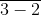)

Solution:

It is given that

12 1/2 – [8 1/2 + {9 – (5 –)

We can write it as

= 25/2 – [17/2 + {9 – (5 – 1)}]

By further calculation

= 25/2 – [17/2 + {9 – 4}]

So we get

= 25/2 – [17/2 + 5]

It can be written as

= 25/2 – 17/2 – 5/1

LCM of 2 and 1 is 2

= (25 – 17 – 10)/ 2

= (25 – 27)/ 2

We get

= – 2/2

= -1

6. 1 1/5 ÷ {2 1/3 – (5 +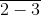)} – 3 ½

Solution:

It is given that

1 1/5 ÷ {2 1/3 – (5 +)} – 3 ½

We can write it as

= 6/5 ÷ {7/3 – (5 – 1)} – 7/2

By further calculation

= 6/5 ÷ {7/3 – 4} – 7/2

LCM of 3 and 1 is 3

= 6/5 ÷ {(7 – 12)/ 3} – 7/2

So we get

= 6/5 ÷ -5/3 – 7/2

It can be write as

= 6/5 × 3/-5 – 7/2

= – 18/25 – 7/2

LCM of 25 and 2 is 50

= (- 36 – 175)/ 50

= – 211/50

= – 4 11/50

7. (1/2 + 2/3) ÷ (3/4 – 2/9)

Solution:

It is given that

(1/2 + 2/3) ÷ (3/4 – 2/9)

LCM of 2 and 3 is 6 and 4 and 9 is 36

= (3 + 4)/ 6 ÷ (27 – 8)/ 36

By further calculation

= 7/6 ÷ 19/36

We can write it as

= 7/6 × 36/19

= 42/19

= 2 4/19

8. 6/5 of (3 1/3 – 2 1/2) ÷ (2 5/21 – 2)

Solution:

It is given that

6/5 of (3 1/3 – 2 1/2) ÷ (2 5/21 – 2)

We can write it as

= 6/5 of (10/3 – 5/2) ÷ (47/21 – 2/1)

LCM of 3 and 2 is 6 and 1 and 21 is 21

= 6/5 of [(20 – 15)/ 6] ÷ [(47 – 42)/ 21]

So we get

= 6/5 of 5/6 ÷ 5/21

= 1 ÷ 5/21

It can be written as

= 1 × 21/5

= 21/5

= 4 1/5

9. 10 1/8 of 4/5 ÷ 35/36 of 20/49

Solution:

It is given that

10 1/8 of 4/5 ÷ 35/36 of 20/49

We can write it as

= 81/8 of 4/5 ÷ 35/36 of 20/49

By further calculation

= 81/10 ÷ 25/63

So we get

= 81/10 × 63/25

= 5103/250

= 20 103/250

10. 5 3/4 – 3/7 × 15 3/4 + 2 2/35 ÷ 1 11/25

Solution:

It is given that

5 3/4 – 3/7 × 15 3/4 + 2 2/35 ÷ 1 11/25

We can write it as

= 23/4 – 3/7 × 63/4 + 72/35 ÷ 36/25

By further calculation

= 23/4 – 3/7 × 63/4 + 72/35 × 25/36

So we get

= 23/4 – 27/4 + 10/7

LCM of 4 and 7 is 28

= (161 – 189 + 40)/ 28

= 12/28

= 3/7

11. 3/4 of 7 3/7 – 5 3/5 ÷ 3 4/15

Solution:

It is given that

3/4 of 7 3/7 – 5 3/5 ÷ 3 4/15

We can write it as

= 3/4 of 52/7 – 28/5 ÷ 49/15

By further calculation

= 39/7 – 28/5 ÷ 49/15

So we get

= 39/7 – 28/5 × 15/49

By multiplication

= 39/7 – 12/7

= (39 – 12)/ 7

= 27/7

= 3 6/7

#### Exercise 3E page: 52

1. A line AB is of length 6 cm. Another line CD is of length 15 cm. What fraction is:

(i) the length of AB to that of CD?

(ii) ½ the length of AB to that of 1/3 of CD?

(iii) 1/5 of CD to that of AB?

Solution:

It is given that

Length of AB = 6 cm

Length of CD = 15 cm

(i) The length of AB to that of CD

= 6/15

= 2/5

(ii) ½ the length of AB to that of 1/3 of CD

1/2 of AB = 1/2 × 6 = 3 cm

1/3 of CD = 1/3 × 15 = 5 cm

So ½ the length of AB to that of 1/3 of CD = 3/5

(iii) 1/5 of CD to that of AB

1/5 of CD = 1/5 × 15 = 3 cm

1/5 of CD to that of AB = 3/6 = 1/2

2. Subtract (2/7 – 5/21) from the sum of 3/4, 5/7 and 7/12.

Solution:

We can write it as

(3/4 + 5/7 + 7/12) – (2/7 – 5/21)

LCM of 4, 7 and 12 is 84 and 7 and 21 is 21

= [(63 + 60 + 49)/ 84] – [(6 – 5)/ 21]

By further calculation

= 172/84 – 1/21

LCM of 21 and 84 is 84

= (172 – 4)/ 84

= 168/ 84

= 2

3. From a sack of potatoes weighing 120 kg, a merchant sells portions weighing 6 kg, 5 ¼ kg, 9 ½ kg and 9 ¾ kg respectively.

(i) How many kg did he sell?

(ii) How many kg are still left in the sack?

Solution:

Weight of potato = 120 kg

(i) Potatoes sold by merchant = 6 kg + 5 ¼ kg + 9 ½ kg + 9 ¾ kg

It can be written as

= (6 + 21/4 + 19/2 + 39/4) kg

LCM of 1, 4 and 2 is 4

= (24 + 21 + 38 + 39)/ 4

By further calculation

= 122/4

= 61/2 kg

= 30 ½ kg

(ii) Potatoes left in the sack = 120 kg – 30 ½ kg

It can be written as

= (120/ 1 – 61/2) kg

By further calculation

= (240 – 61)/ 2

So we get

= 179/2 kg

= 89 ½ kg

4. If a boy works for six consecutive days for 8 hours, 7 ½ hours, 8 ¼ hours, 6 ¼ hours, 6 ¾ hours and 7 hours respectively, how much money will he earn at the rate of ₹ 36 per hour?

Solution:

Hours worked by a boy for six consecutive days = 8 hours + 7 ½ hours + 8 ¼ hours + 6 ¼ hours + 6 ¾ hours + 7 hours

It can be written as

= (8 + 15/2 + 33/4 + 25/4 + 27/4 + 7) hours

LCM of 2 and 4 is 4

= (32 + 30 + 33 + 25 + 27 + 28)/ 4 hours

So we get

= 175/4 hours

= 43 ¾ hours

He earned ₹ 36 per hour

So the total earnings = 175/4 × 36

We get

= 175 × 9

= ₹ 1575

5. A student bought 4 1/3 m of yellow ribbon, 6 1/6 m of red ribbon and 3 2/9 m of blue ribbon decorating a room. How many metres of ribbon did he buy?

Solution:

It is given that

Length of yellow ribbon = 4 1/3 m = 13/3 m

Length of red ribbon = 6 1/6 m = 37/6 m

Length of blue ribbon = 3 2/9 m = 29/9 m

So the total length = 13/3 + 37/6 + 29/9

LCM of 3, 6 and 9 is 18

= (78 + 111 + 58)/ 18

So we get

= 247/ 18

= 13 13/18 m

6. In a business, Ram and Deepak invest 3/5 and 2/5 of the total investment. If ₹ 40, 000 is the total investment, calculate the amount invested by each.

Solution:

It is given that

Total investment = ₹ 40, 000

Ram’s investment = 3/5 of ₹ 40, 000

It can be written as

= 3/5 × 40, 000

By further calculation

= 3 × 8000

= ₹ 24, 000

Deepak’s investment = 2/5 of ₹ 40, 000

It can be written as

= 2/5 × 40, 000

By further calculation

= 2 × 8000

= ₹ 16, 000

7. Geeta had 30 problems for home work. She worked out 2/3 of them. How many problems were still left to be worked out by her?

Solution:

Number of problems Geeta had for home work = 30

Number of problems worked out by Geeta = 2/3 of 30

We can write it as

= 2/3 × 30

= 20

Number of problems still left to be worked out by her = 30 – 20 = 10

8. A picture was marked at ₹ 90. It was sold at 3/4 of its marked price. What was the sale price?

Solution:

It is given that

Marked price of picture = ₹ 90

Sale price of picture = ¾ of ₹ 90

We can write it as

= ¾ × 90

= ₹ 270/4

= ₹ 67 ½

= ₹ 67.50

9. Mani had sent fifteen parcels of oranges. What was the total weight of the parcels, if each weighed 10 ½ kg?

Solution:

Number of parcels = 15

Weight of each parcel = 10 ½ kg = 21/2 kg

So the total weight of parcels = 15 of 21/2 kg

We can write it as

= 21/2 × 15 kg

By further calculation

= 315/2

= 157 ½ kg

= 157.5 kg

10. A rope is 25 ½ m long. How many pieces each of 1 ½ m length can be cut out from it?

Solution:

Length of rope = 25 ½ m = 51/2 m

Length of each piece = 1 ½ m = 3/2 m

So the number of pieces = 51/2 ÷ 3/2

We can write it as

= 51/2 × 2/3

= 17 pieces

11. The heights of two vertical poles, above the earth’s surface, are 14 ¼ m and 22 1/3 m respectively. How much higher is the second pole as compared with the height of the first pole?

Solution:

It is given that

Height of first pole above the earth’s surface = 14 ¼ m

Height of second pole above the earth’s surface = 22 1/3 m

So height of second pole when compared to first pole = 22 1/3 – 14 1/4

We can write it as

= 67/3 – 57/4

LCM of 3 and 4 is 12

= (268 – 171)/ 12

By further calculation

= 97/12 m

= 8 1/12 m

12. Vijay weighed 65 ½ kg. He gained 1 2/5 kg during the first week, 1 ¼ kg during the second week, but lost 5/16 kg during the third week. What was his weight after the third week?

Solution:

It is given that

Weight of Vijay = 65 ½ kg

Weight gained during first week = 1 2/5 kg

Weight gained during second week = 1 ¼ kg

Weight lost during third week = 5/16 kg

So the weight of Vijay after third week = 65 ½ + 1 2/5 + 1 ¼ – 5/16

It can be written as

= 131/2 + 7/5 + 5/4 – 5/16

LCM of 2, 5, 4 and 16 is 8-

= (5240 + 112 + 100 – 25)/ 80

By further calculation

= (5452 – 25)/ 80

= 5427/80 kg

= 67 67/80 kg

13. A man spends 2/5 of his salary on food and 3/10 on house rent, electricity, etc. What fraction of his salary is still left with him?

Solution:

Consider salary of man = ₹ 1

Salary spent on food = 2/5 of ₹ 1 = ₹ 2/5

Salary spent on house rent = 3/10 of ₹ 1 = ₹ 3/10

So the total salary spent = 2/5 + 3/10

LCM of 5 and 10 is 10

= (4 + 3)/ 10

= 7/10

Salary still left with him = 1 – 7/10

LCM of 1 and 10 is 10

= (10 – 7)/ 10

= 3/10

14. A man spends 2/5 of his salary on food and 3/10 of the remaining on house rent, electricity, etc. What fraction of his salary is still left with him?

Solution:

Consider ₹ 1 as the total salary

Salary spent on food = 2/5 of ₹ 1 = ₹ 2/5

So the remaining salary = 1 – 2/5

It can be written as

= (5 – 2)/ 5

= ₹ 3/5

Salary spent on house rent = 3/10 of 3/5 = ₹ 9/50

So the remaining salary = 3/5 – 9/50

LCM of 5 and 50 is 50

= (30 – 9)/ 50

= ₹ 21/50

15. Shyam bought a refrigerator for ₹ 5,000. He paid 1/10 of the price in cash and the rest in 12 equal monthly instalments. How much had he to pay each month?

Solution:

Total cost of refrigerator = ₹ 5000

Cash paid = 1/10 of ₹ 5000

= 1/10 × 5000

= ₹ 500

So the balance amount = 5000 – 500 = ₹ 4500

Number of instalments = 12

So the amount to be paid each month = 4500 ÷ 12

We can write it as

= 4500 × 1/12

= ₹ 375